## Wednesday, December 26, 2018

2013
(November)
MATHEMATICS
(Major)
Course: 502
(Linear Algebra and Number Theory)
Full Marks: 80
Pass Marks: 32
Time: 3 hours
The figures in the margin indicate full marks for the questions
GROUP – A
(Linear Algebra)
(Marks: 40)

1. Answer the following questions: 1x4=4
1. Under what condition, two systems of linear equations over the same field are said to be equivalent?
2. Write the standard basis for the vector space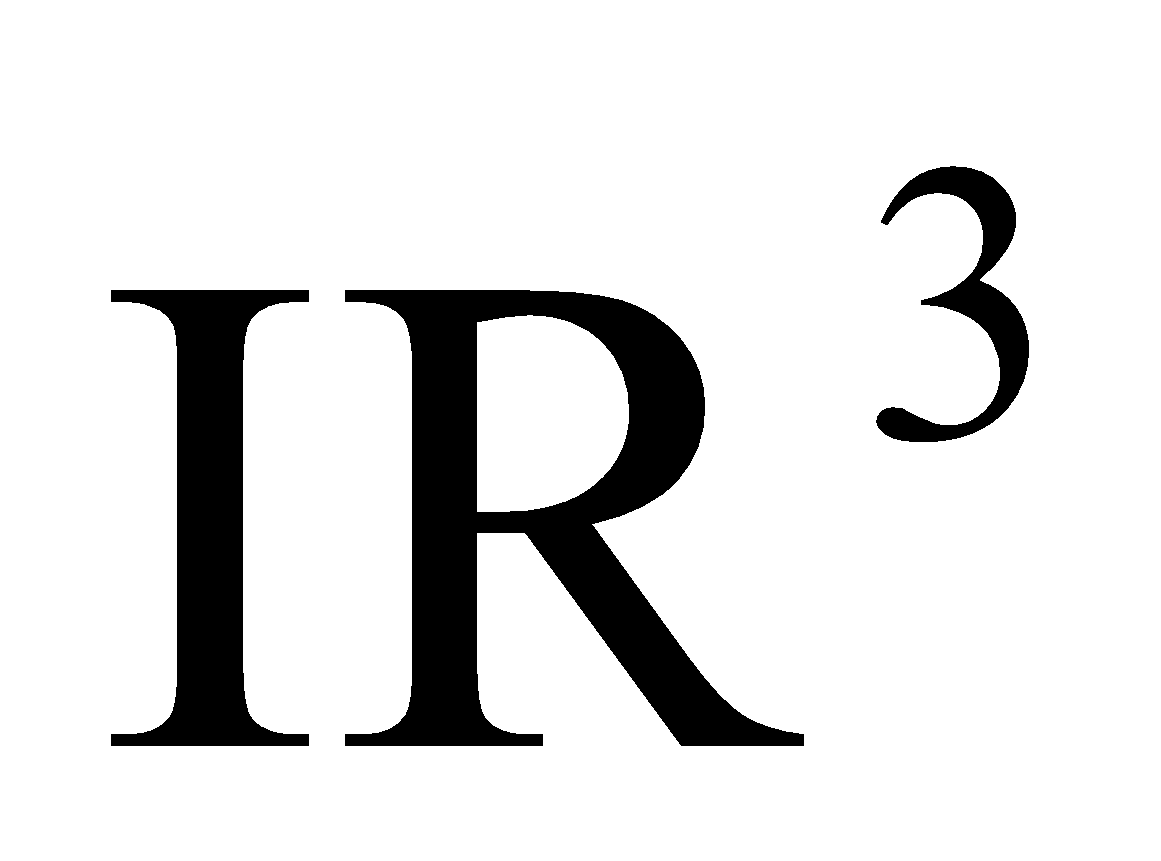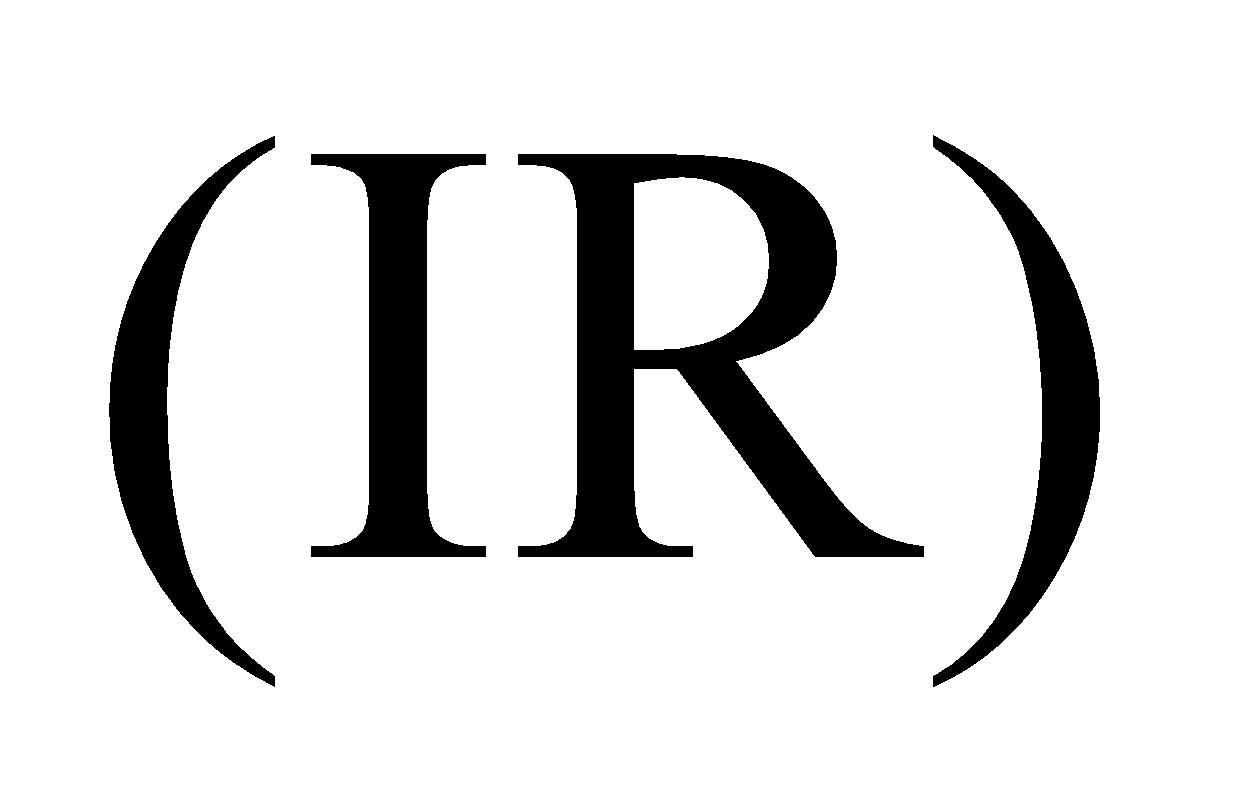.
3. Define null space of a linear transformation.
4. Let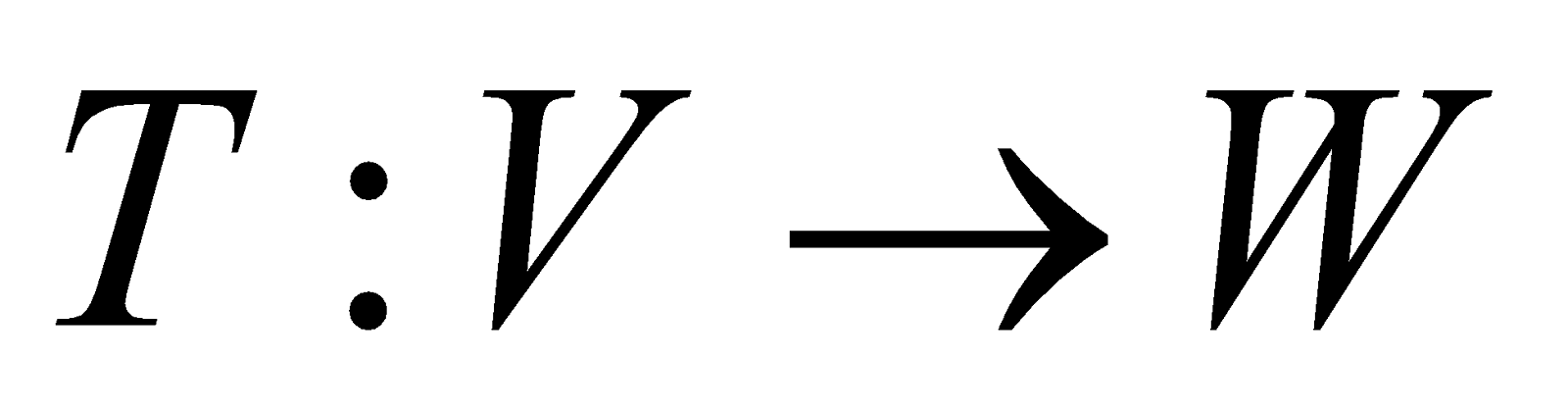be a linear map given by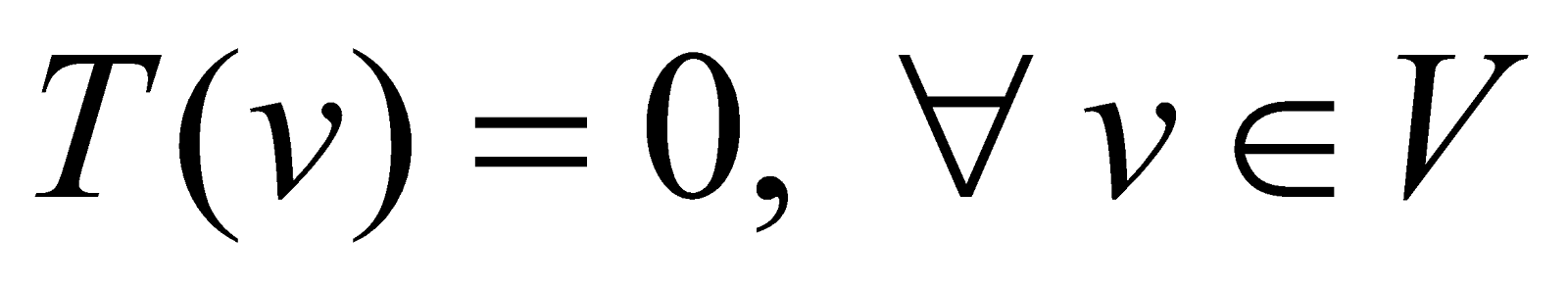. What will be the kernel of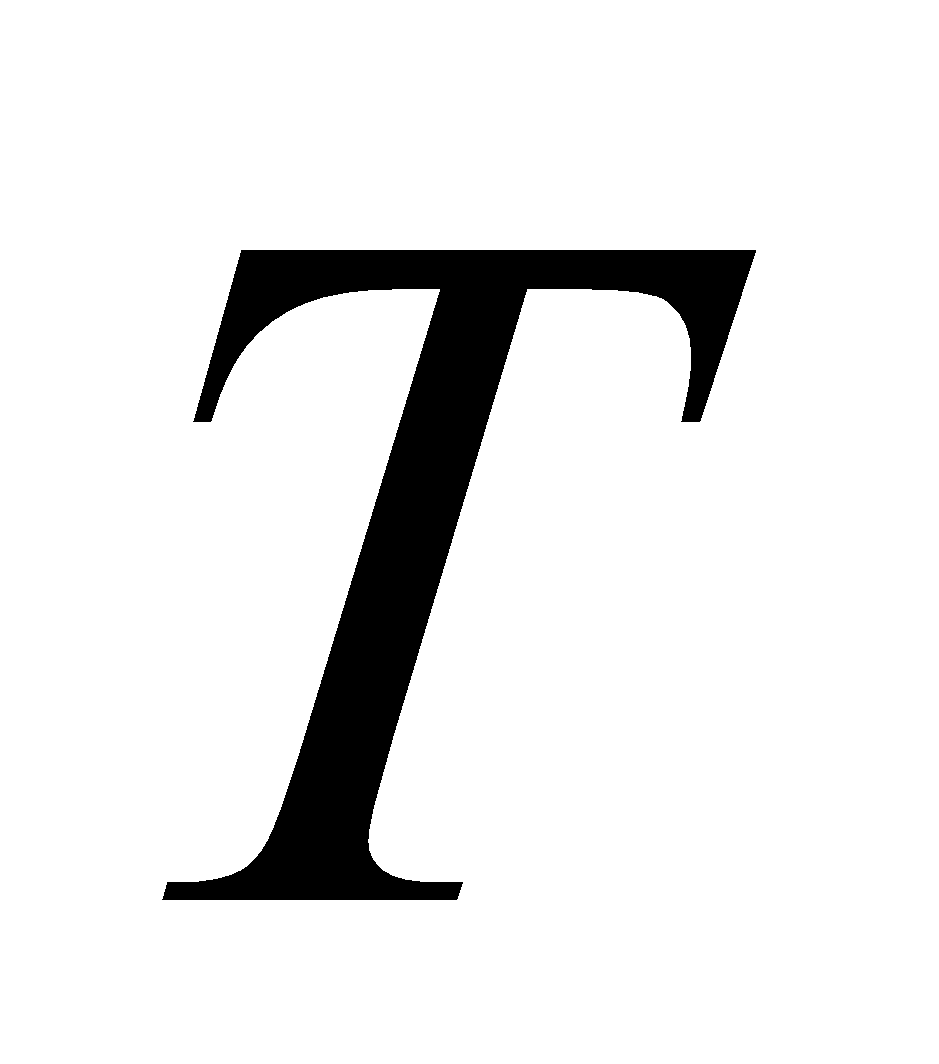?
1. Find the dimension of the quotient space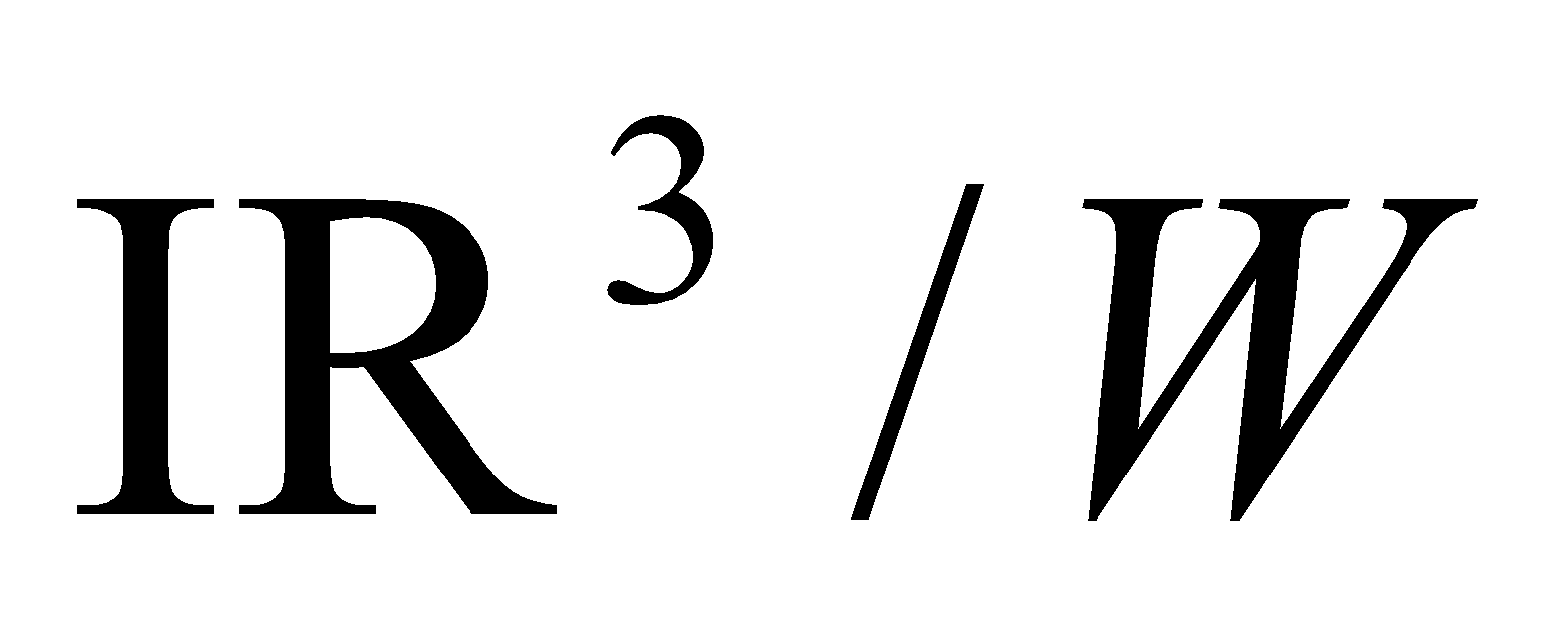, where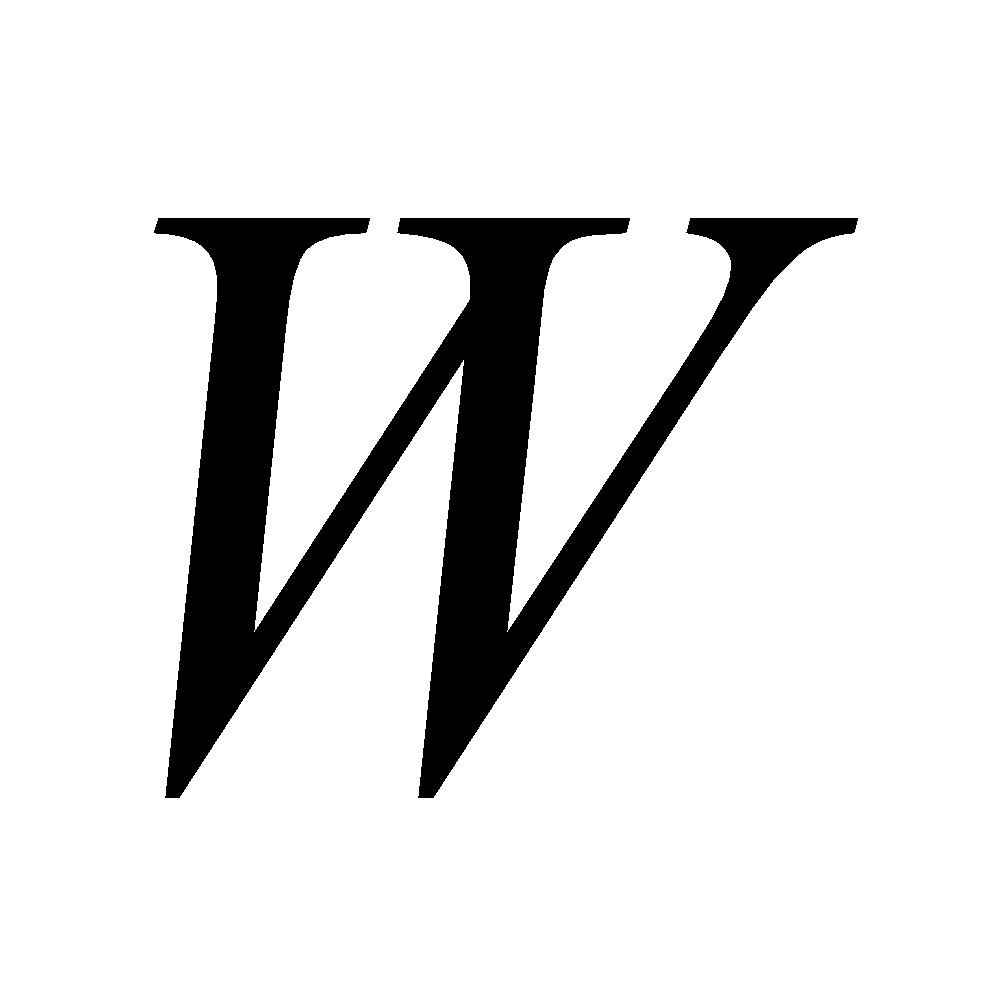is the subspace ofspanned by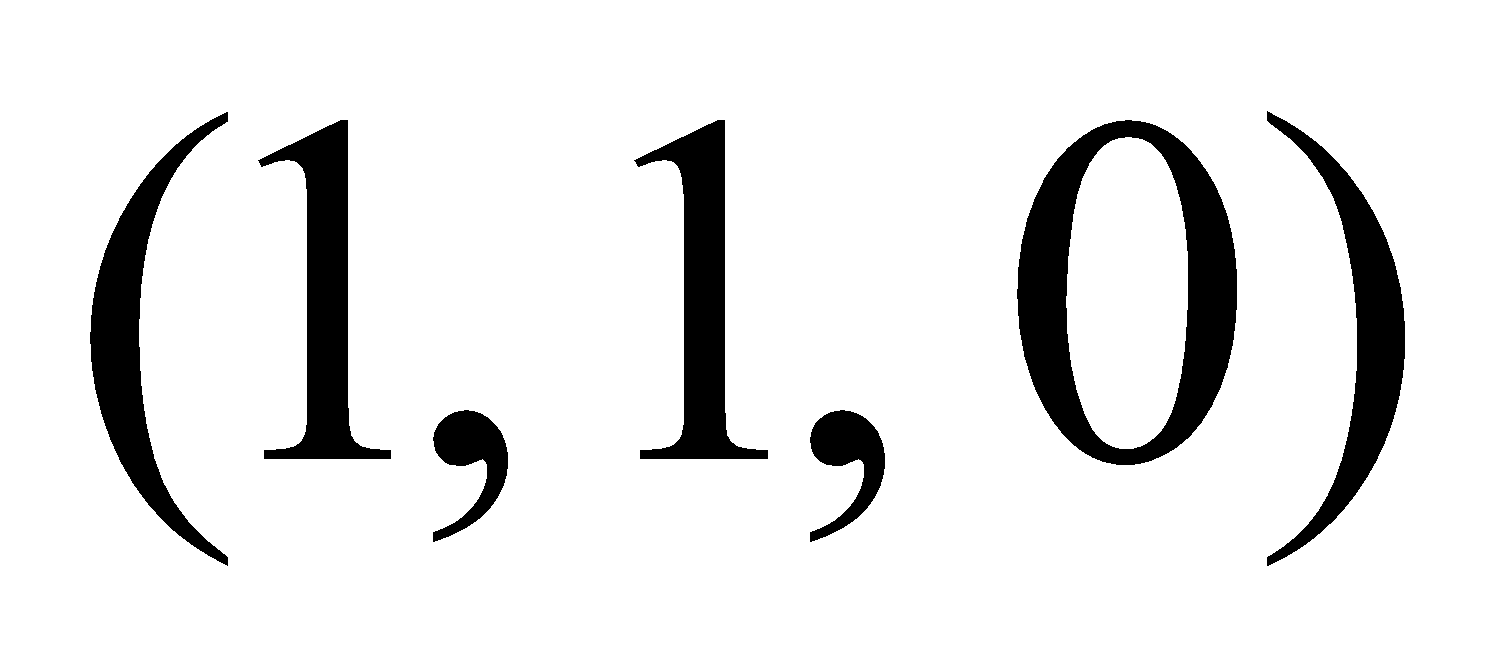and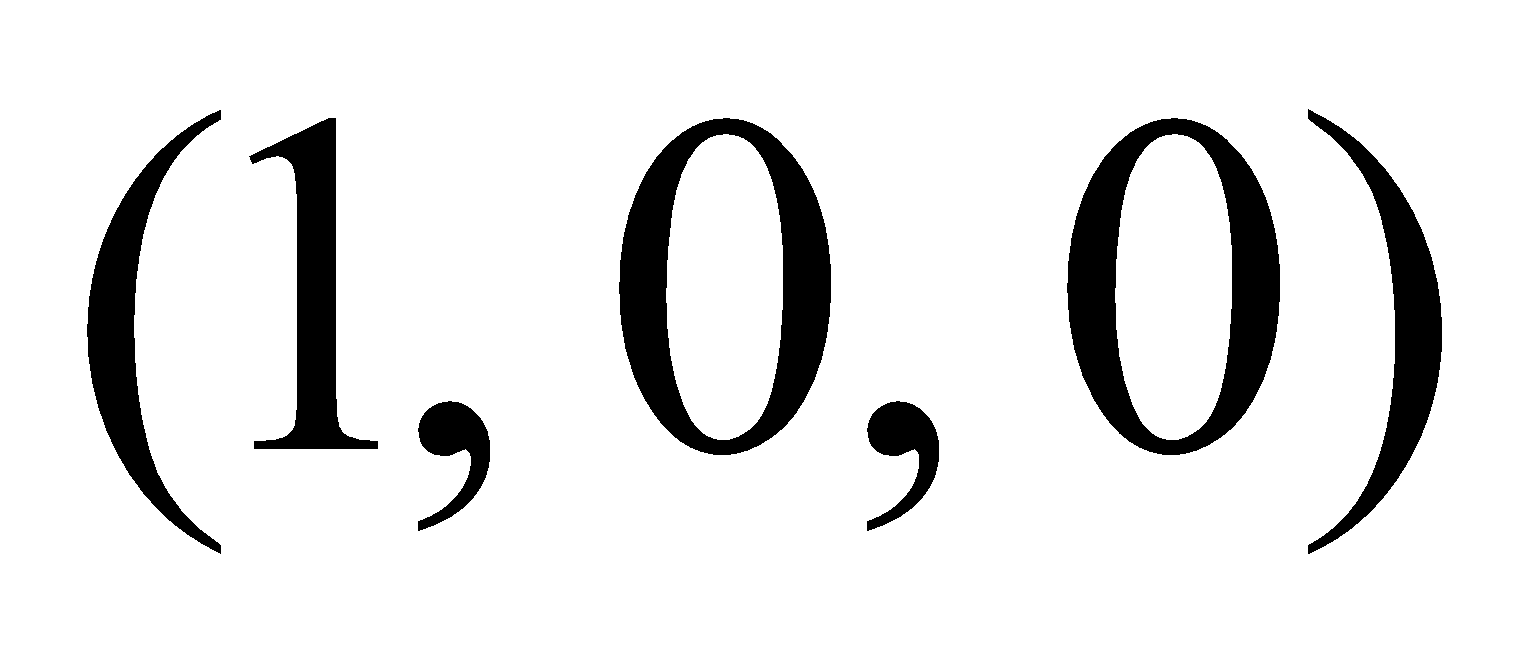. 2
2. If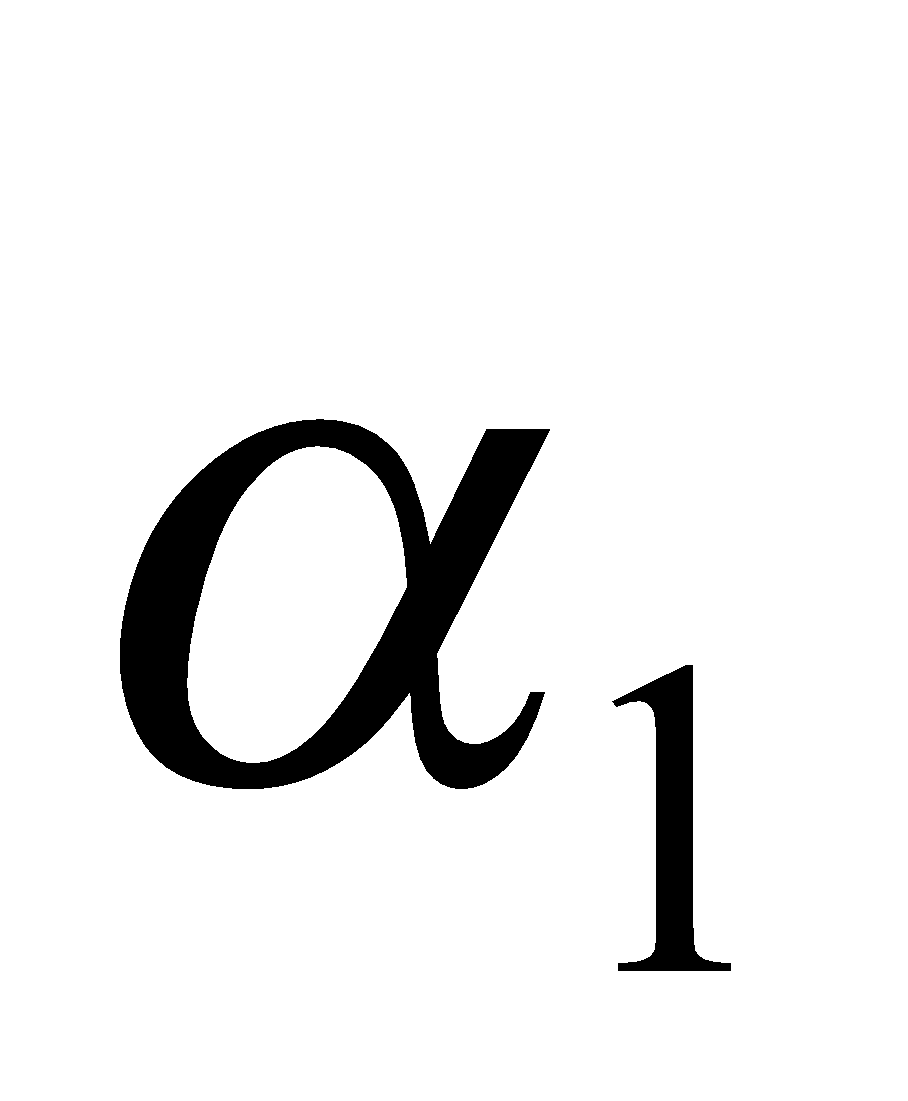and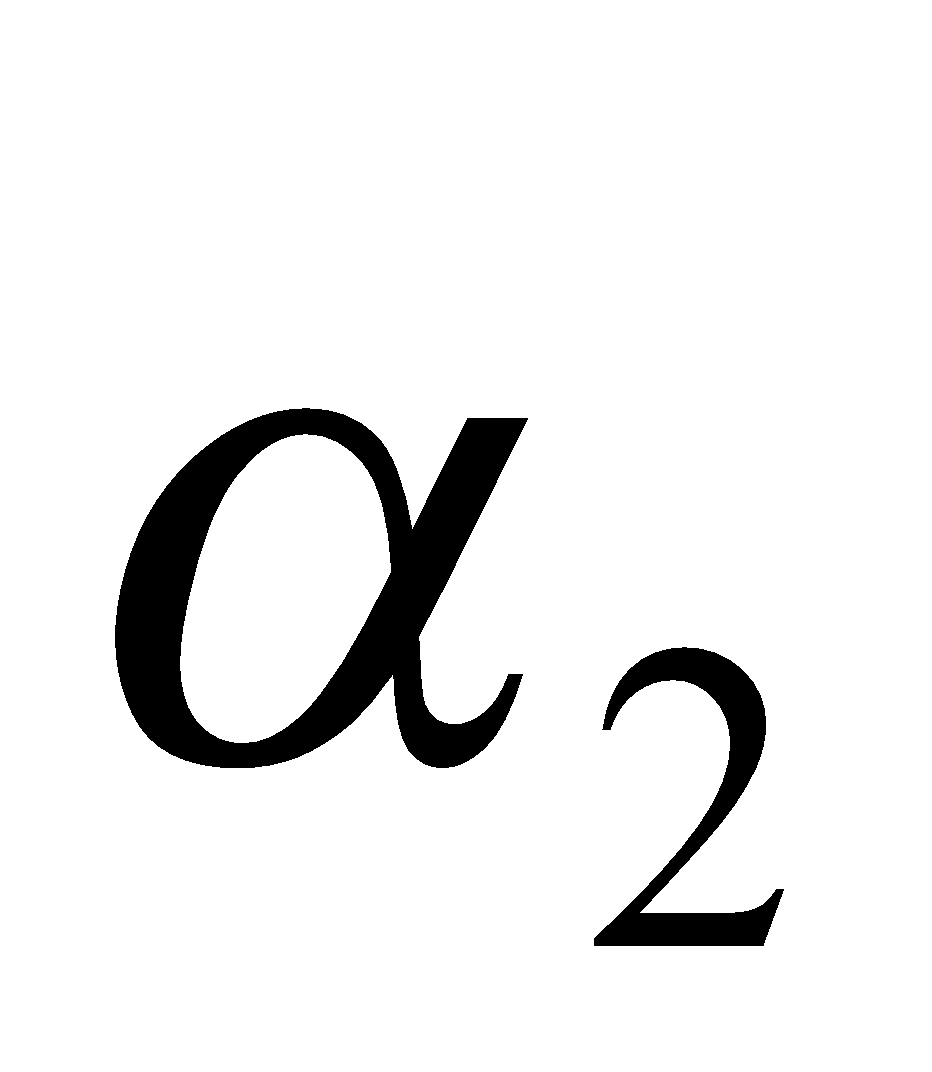are the vectors of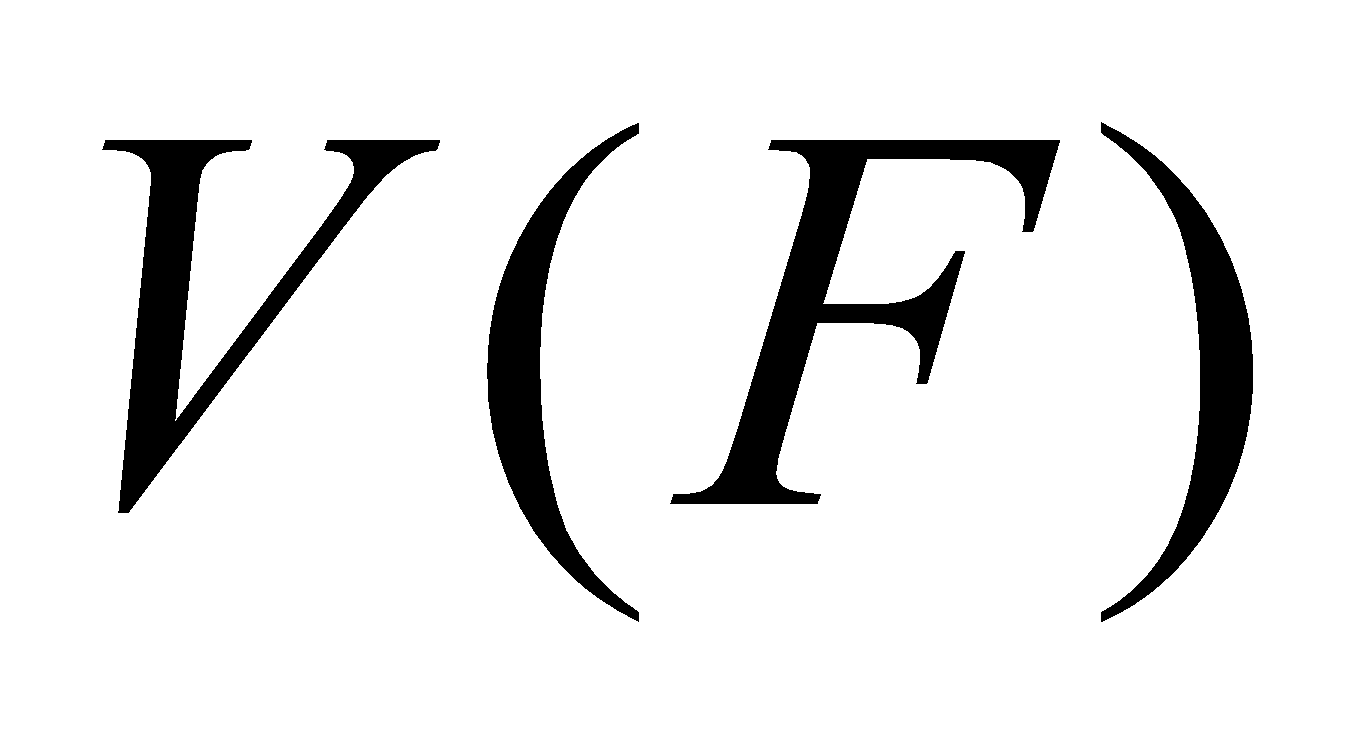and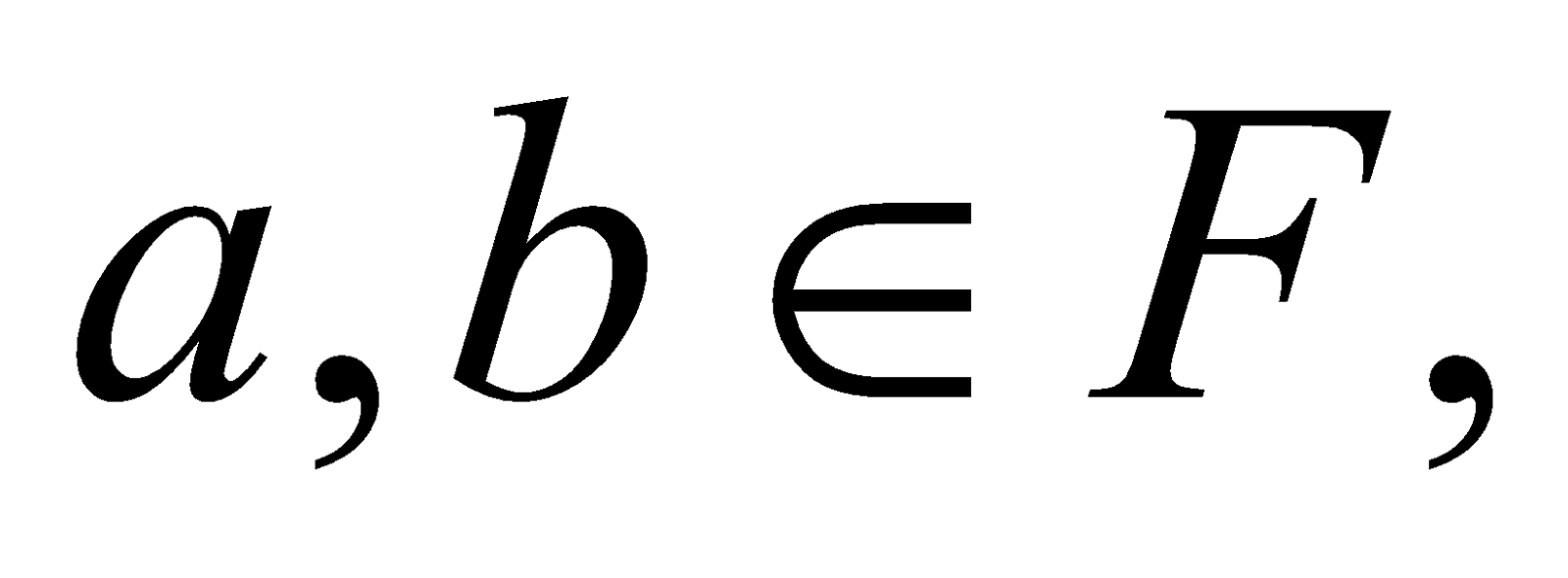then prove that 3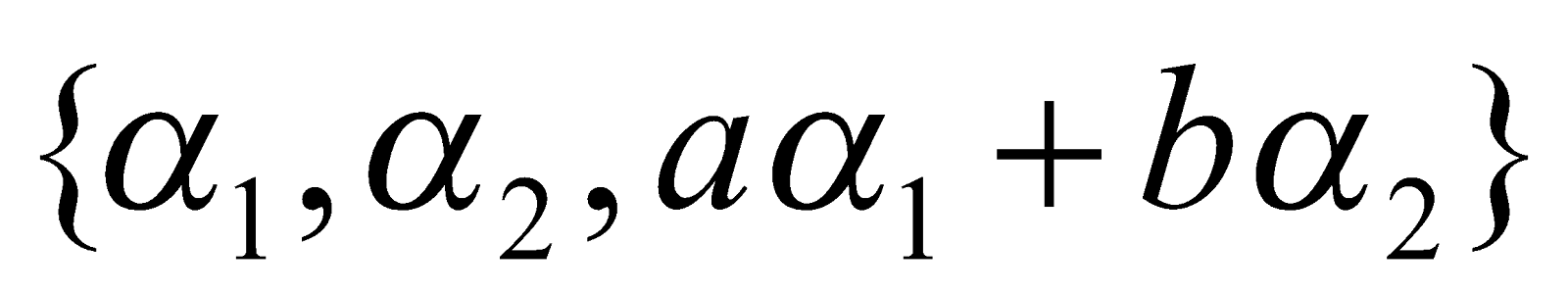is linearly dependent.
1. Show that the subsetofis a subspace of.
1. Prove that the intersection of any two subspaces of a vector space is also a subspace of the vector space.    3
2. Let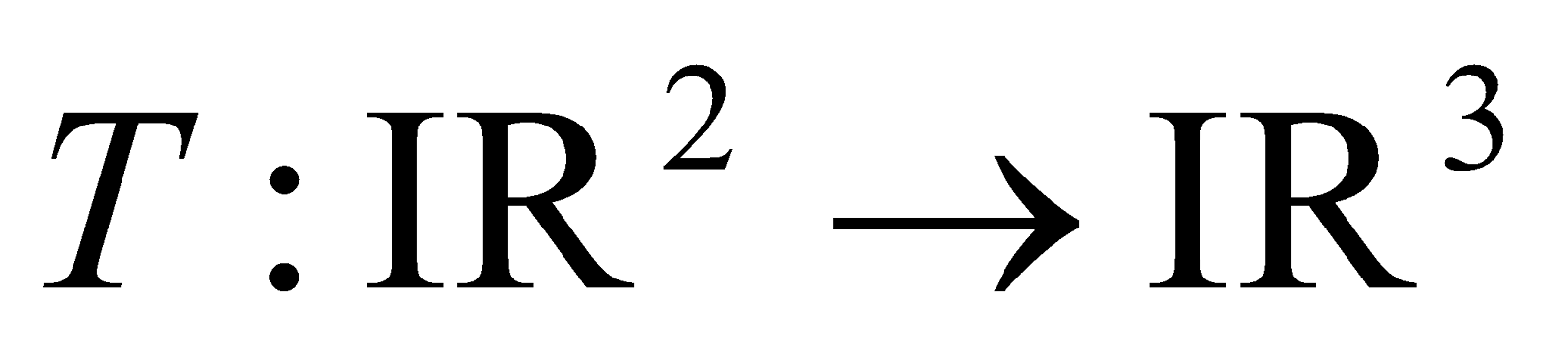be defined by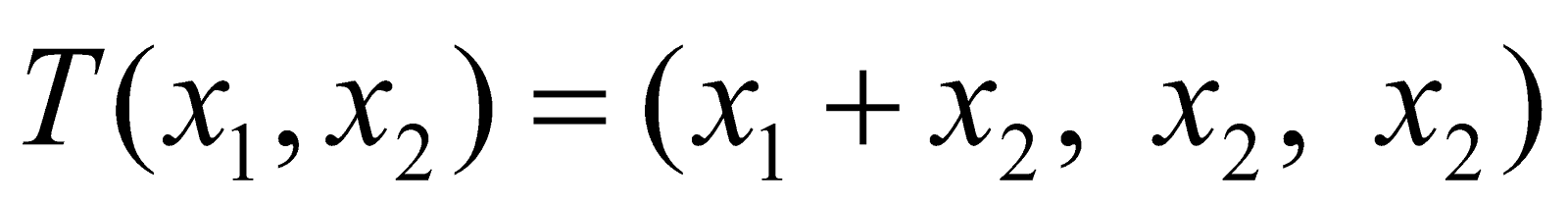Find the matrix of T w.r.t. the standard bases of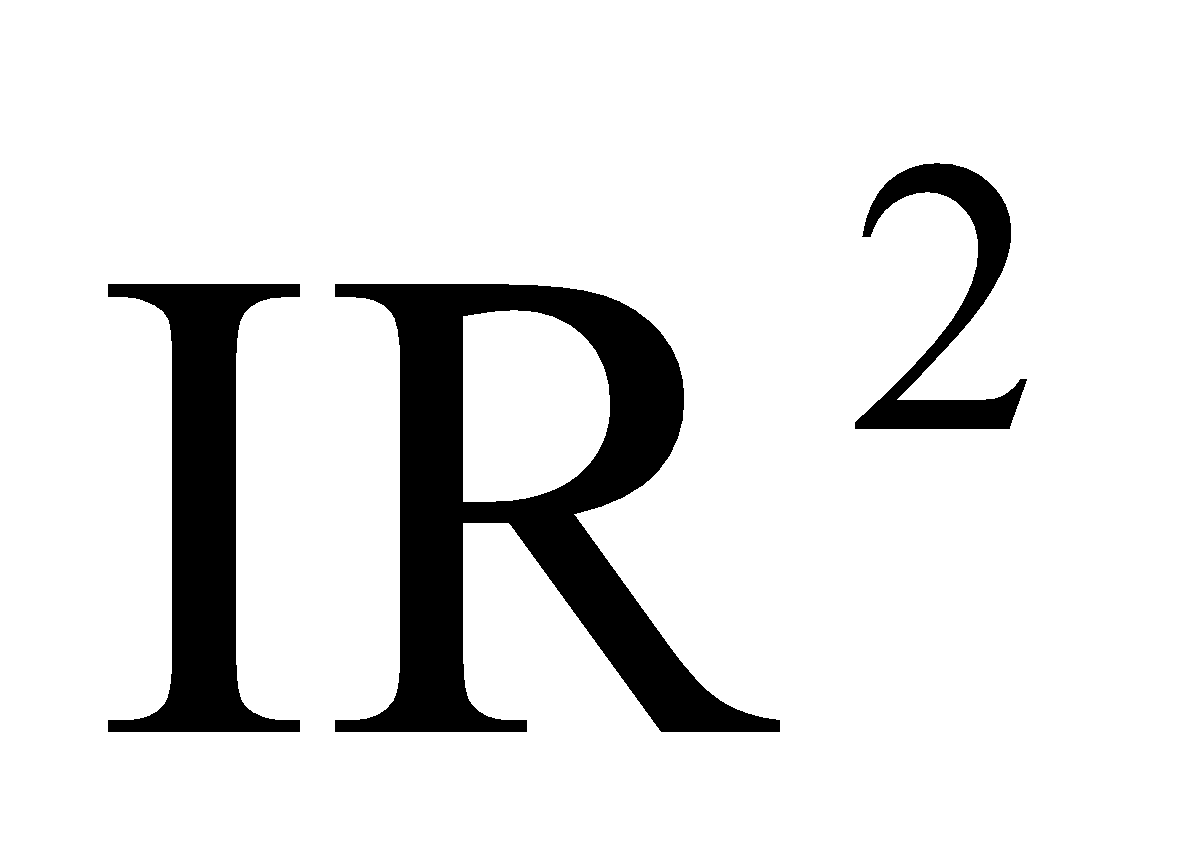andrespectively. 3
1. If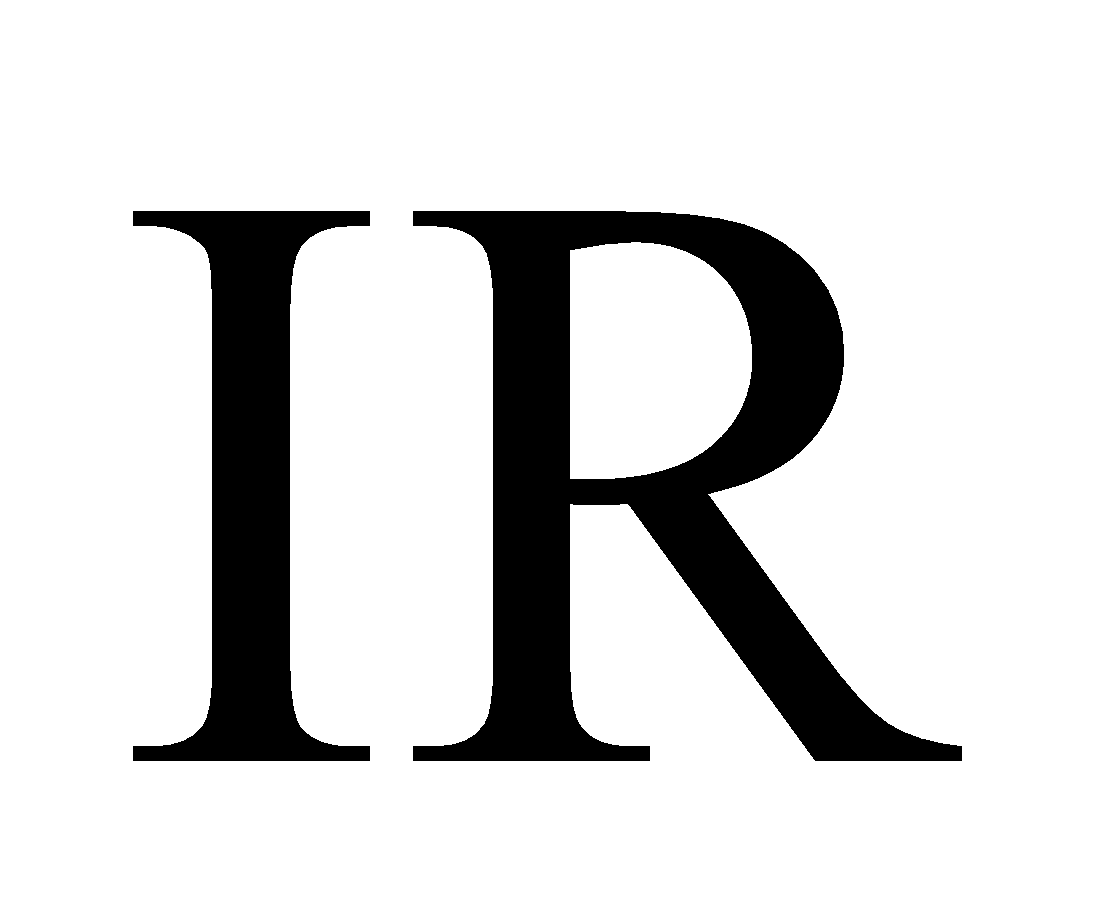be the field of real numbers, then prove that the vectors (a, b) and (c, d) inare linearly dependent if and only if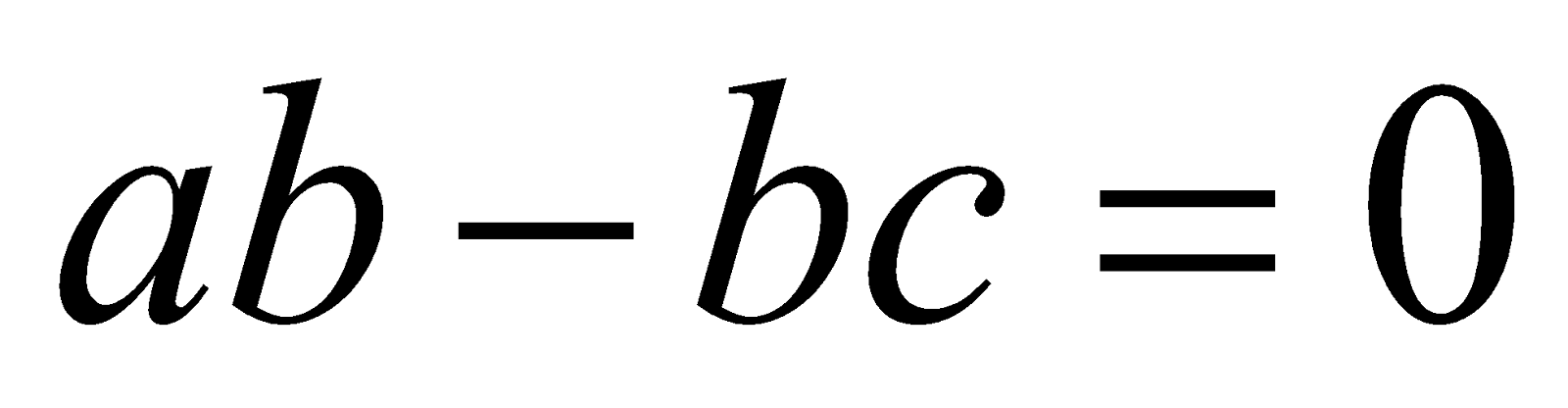. 4

3. Answer any three of the following questions: 6x3=18
1. Define affine space. Letbe a subspace of a vector space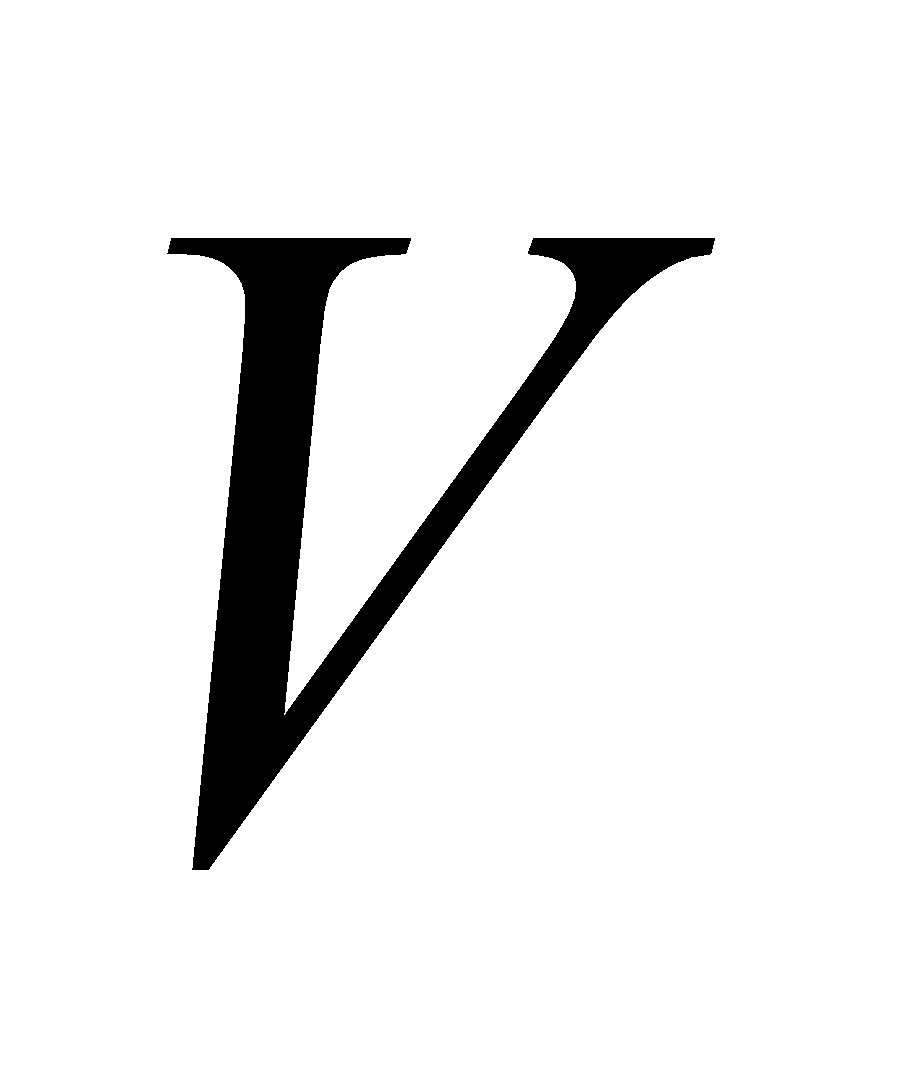and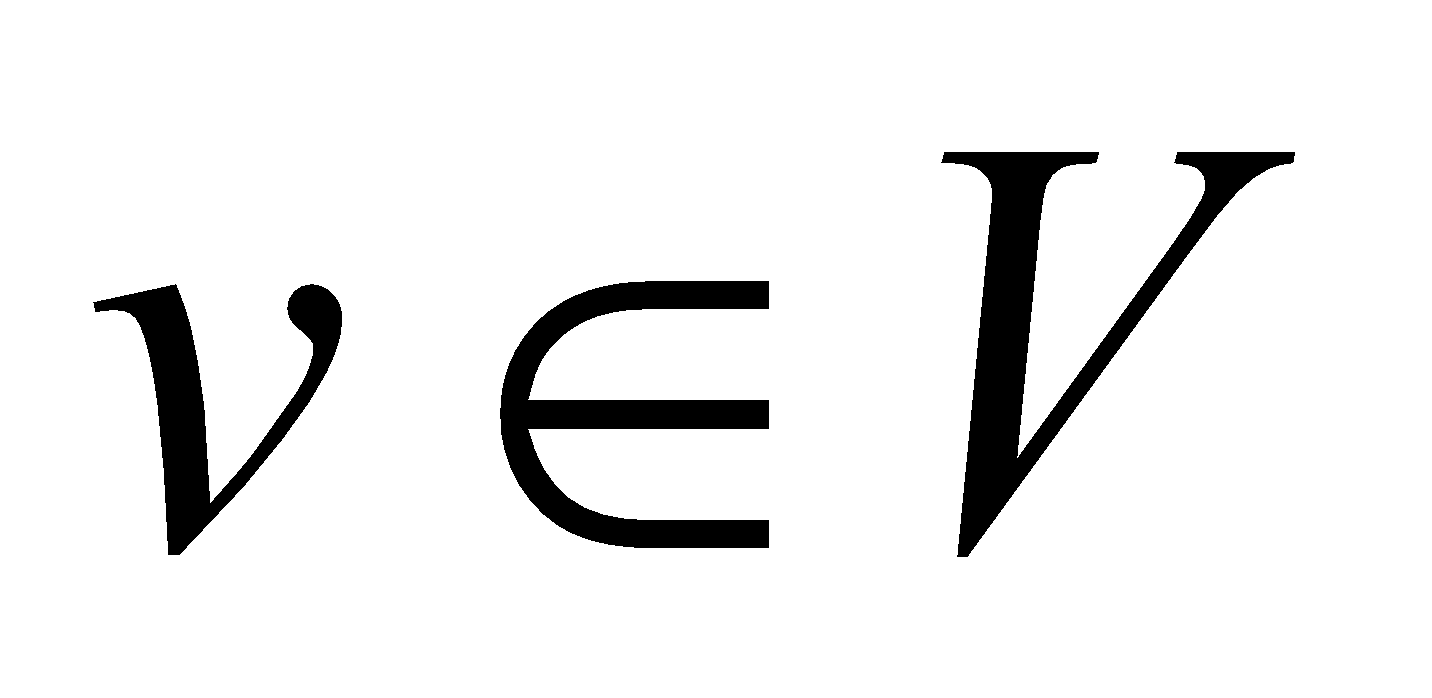be fixed. Prove that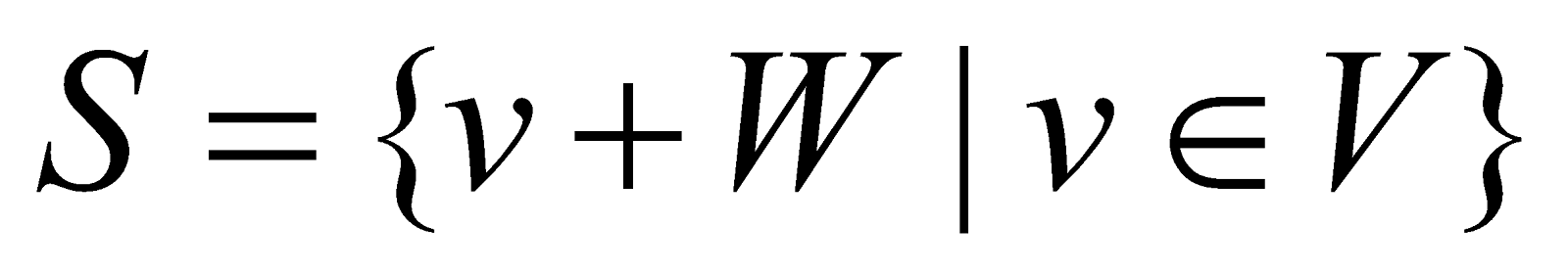is an affine space.
1. Let T be a linear transformation from V into W. then prove that T is non-singular if and only if T carries each linearly independent subset of V onto a linearly independent subset of W.
2. Show that the mappingdefined as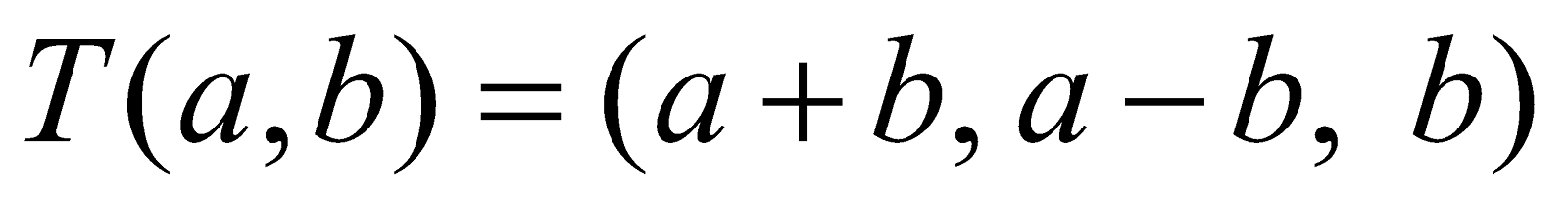is a linear transformation fromto. Find the rank, null space and nullity of T.
3. If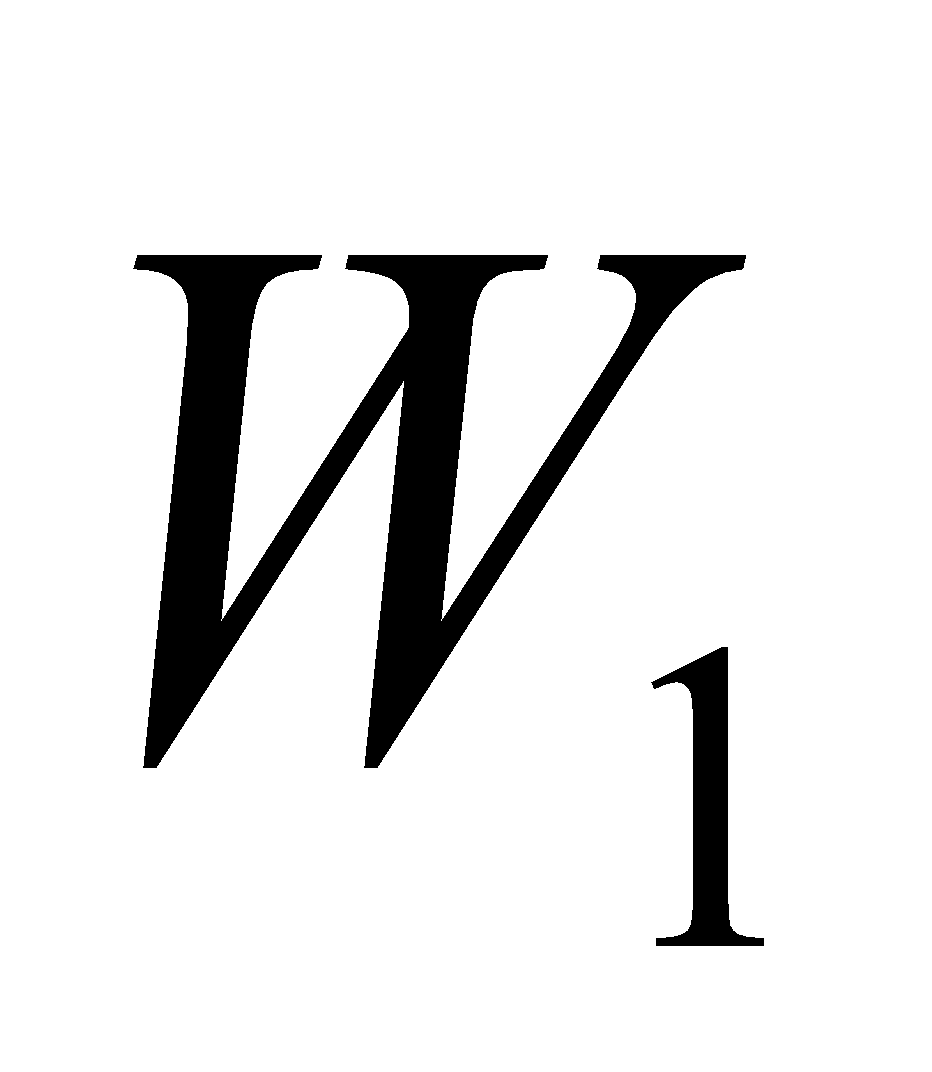and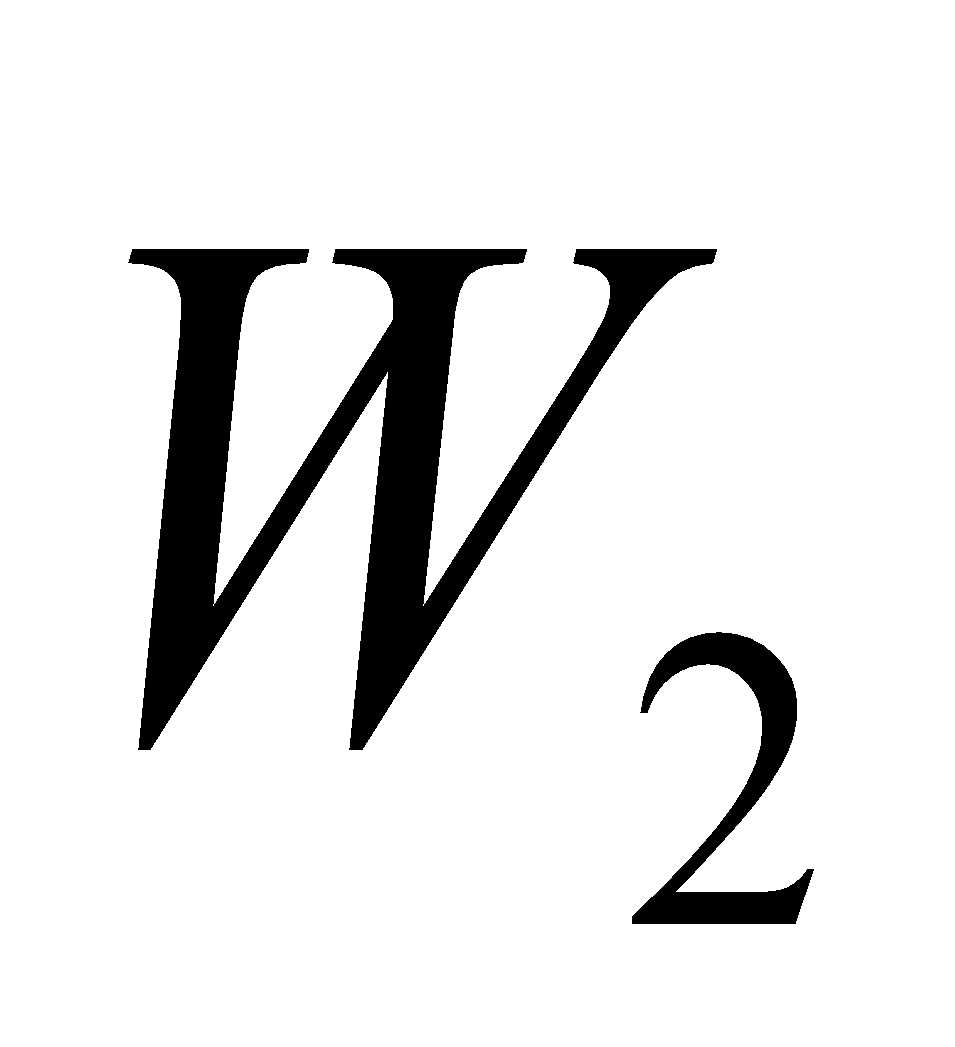are finite-dimensional subspaces of a vector space, then prove that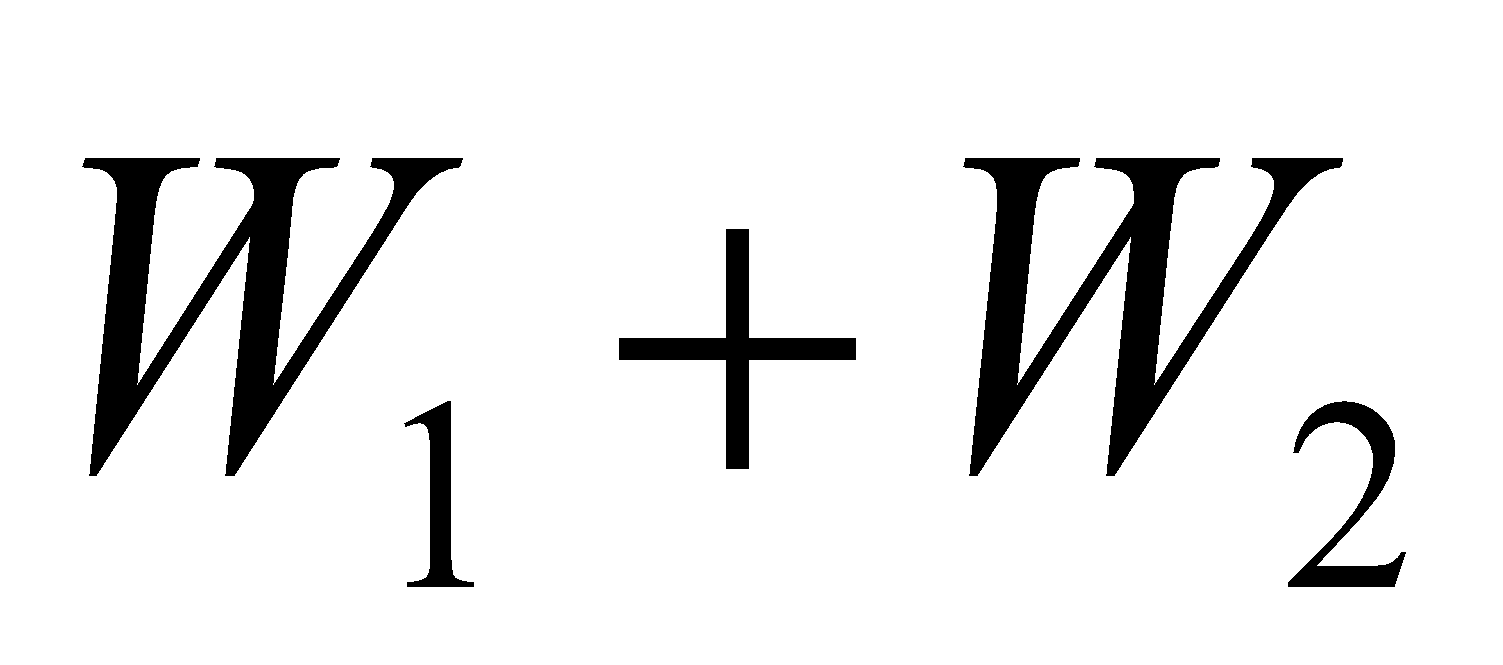is finite-dimensional and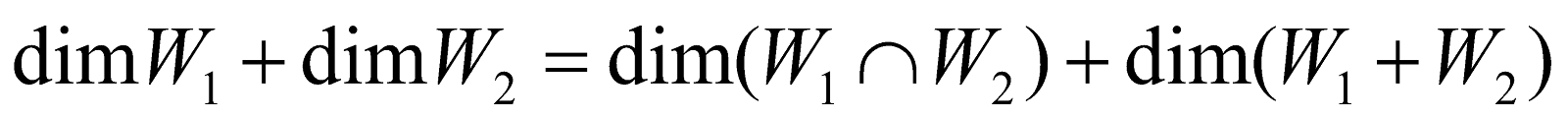GROUP – B
(Number Theory)
(Marks: 40)
4. Answer the following questions: 1x4=4
1. Write the well-ordering principle (WOP) of positive integers.
2. If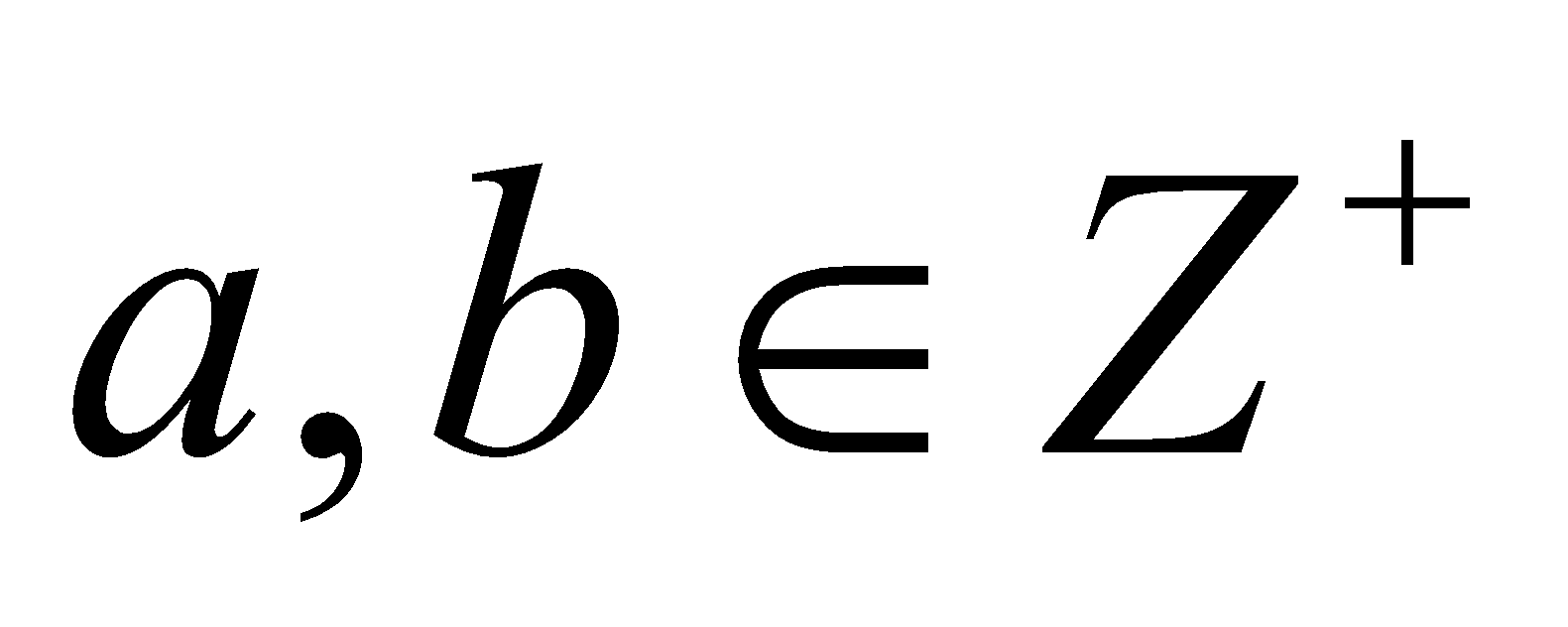and there exists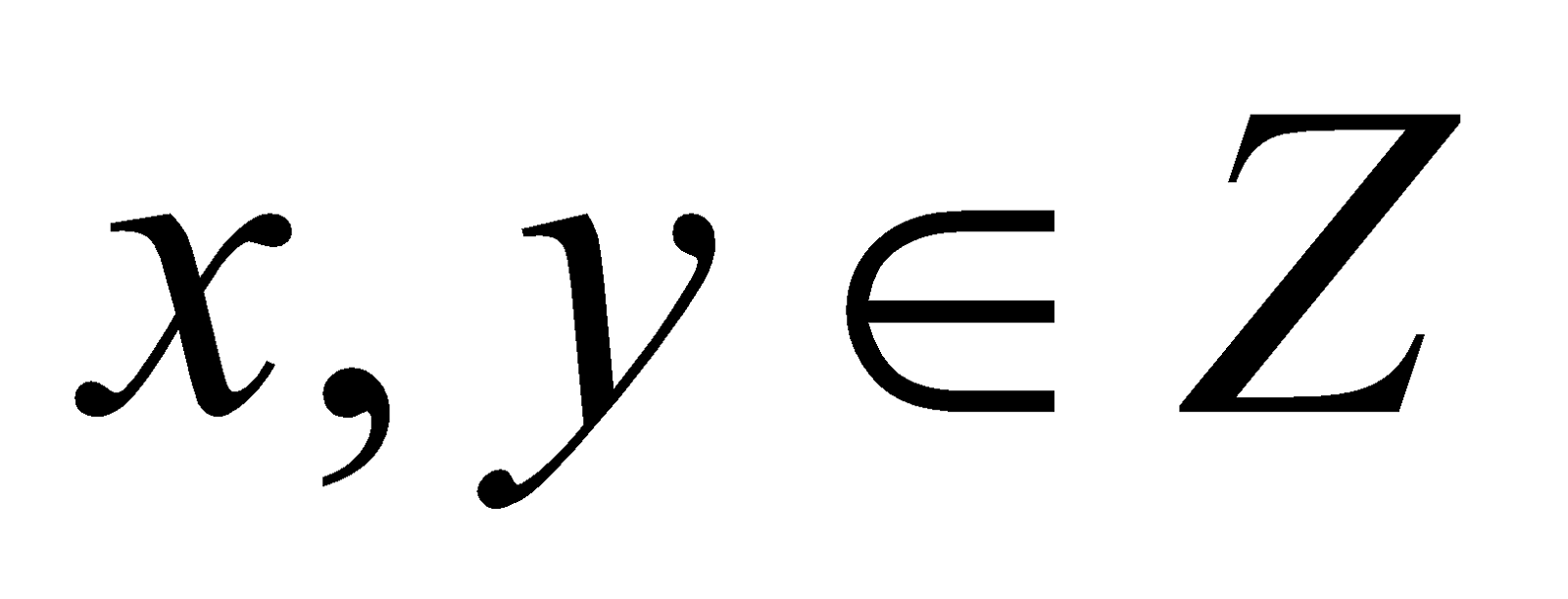such that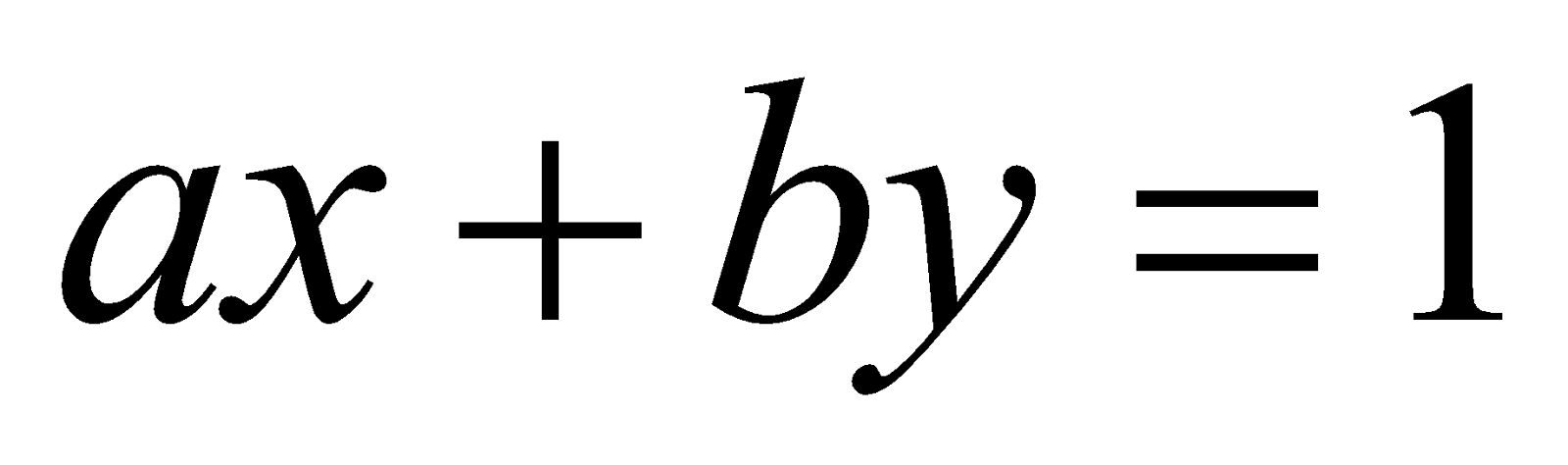, then write the value of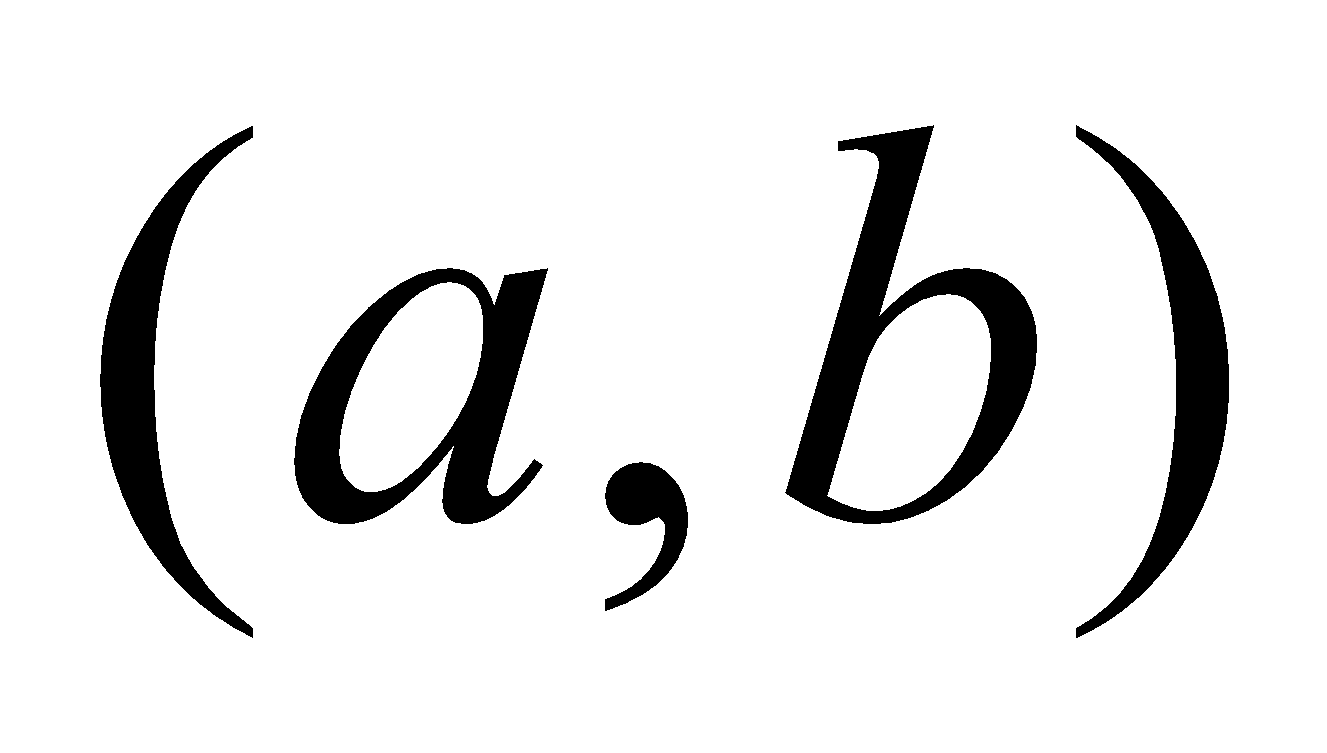.
3. Define Euler’s function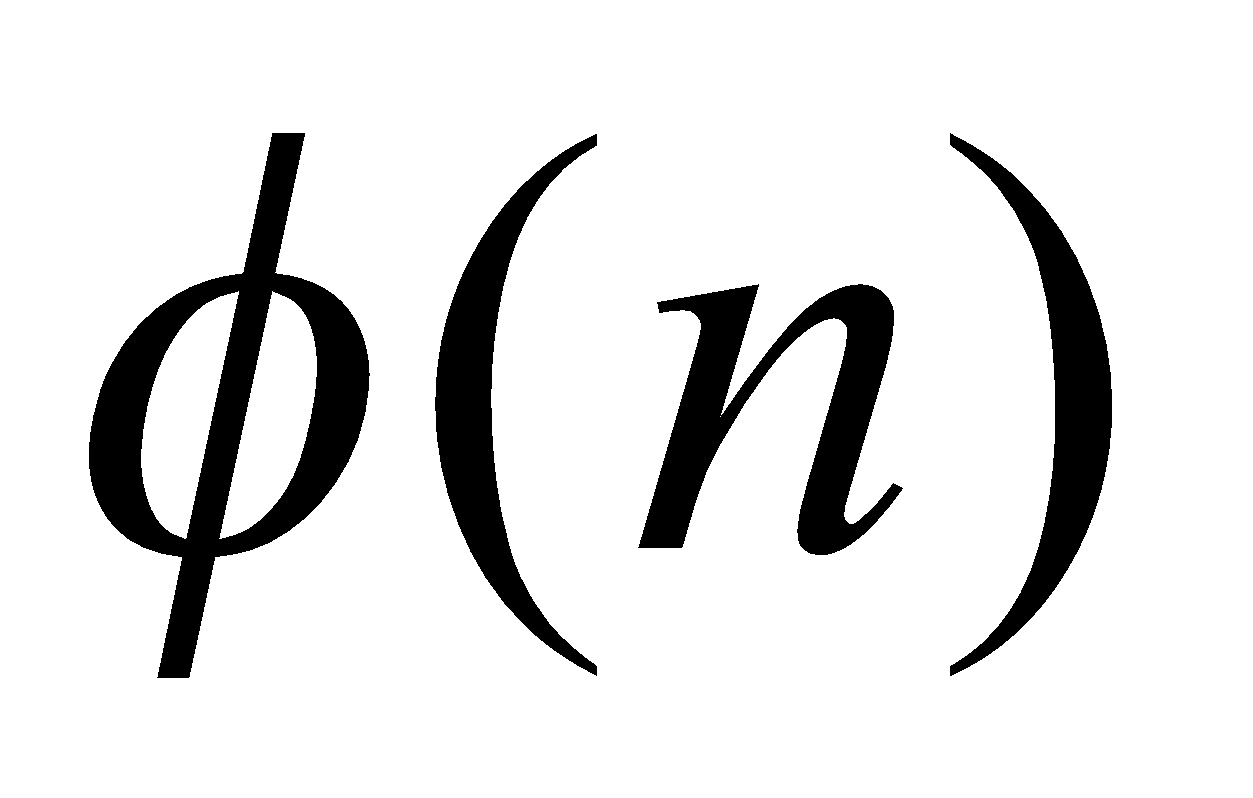.
4. Write a reduced set of residues mod 10.
5. Answer the following questions: 2x3=6
1. Show that the difference between any integer and its cube is always divisible by 6.
2. If g.c.d.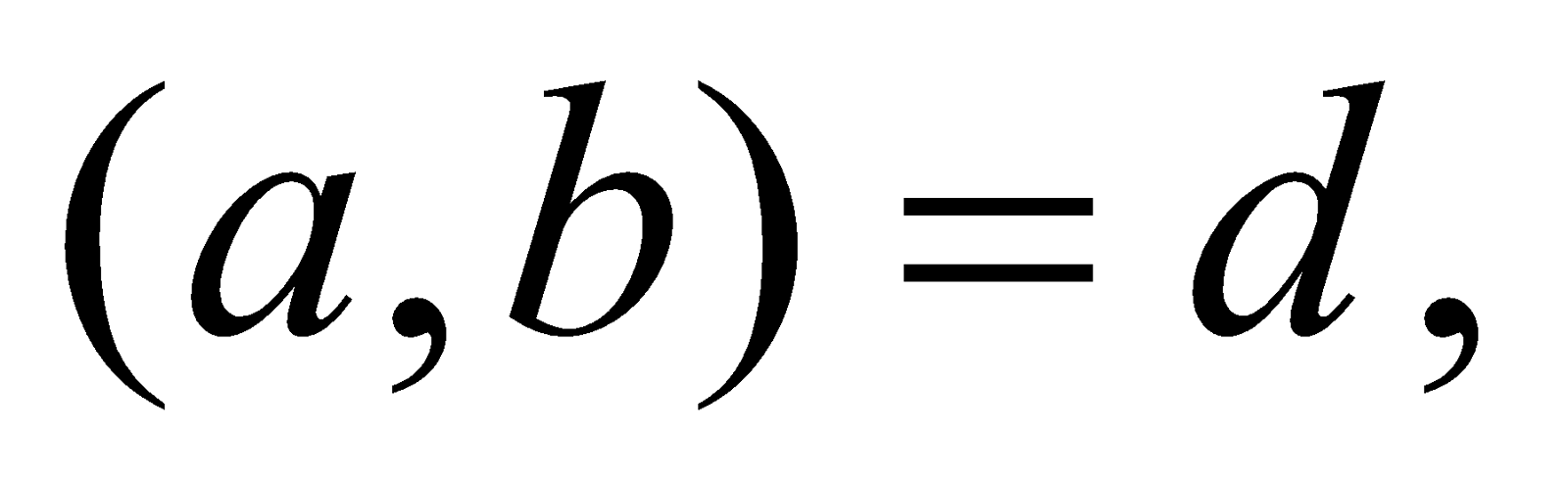then prove that
g.c.d.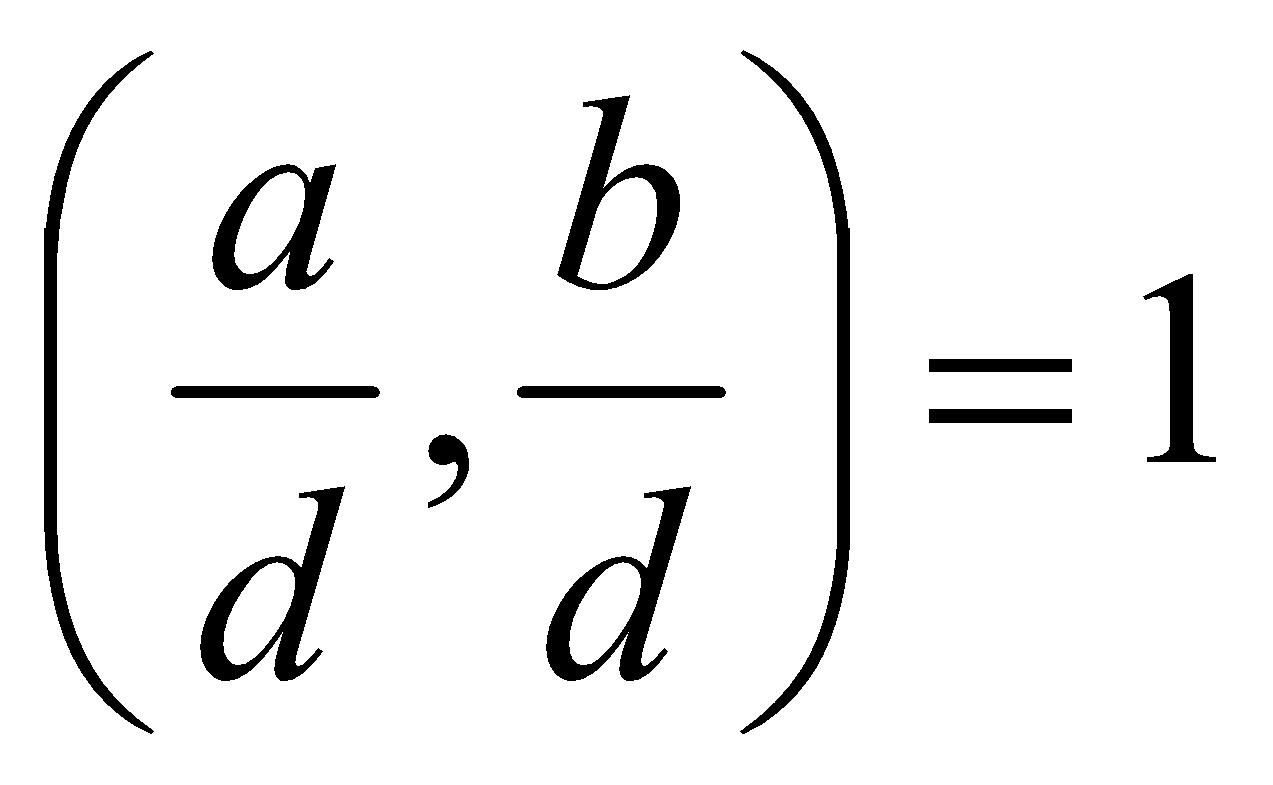1. Under which situation, an arithmetic function is said to be a multiplicative function? Is the function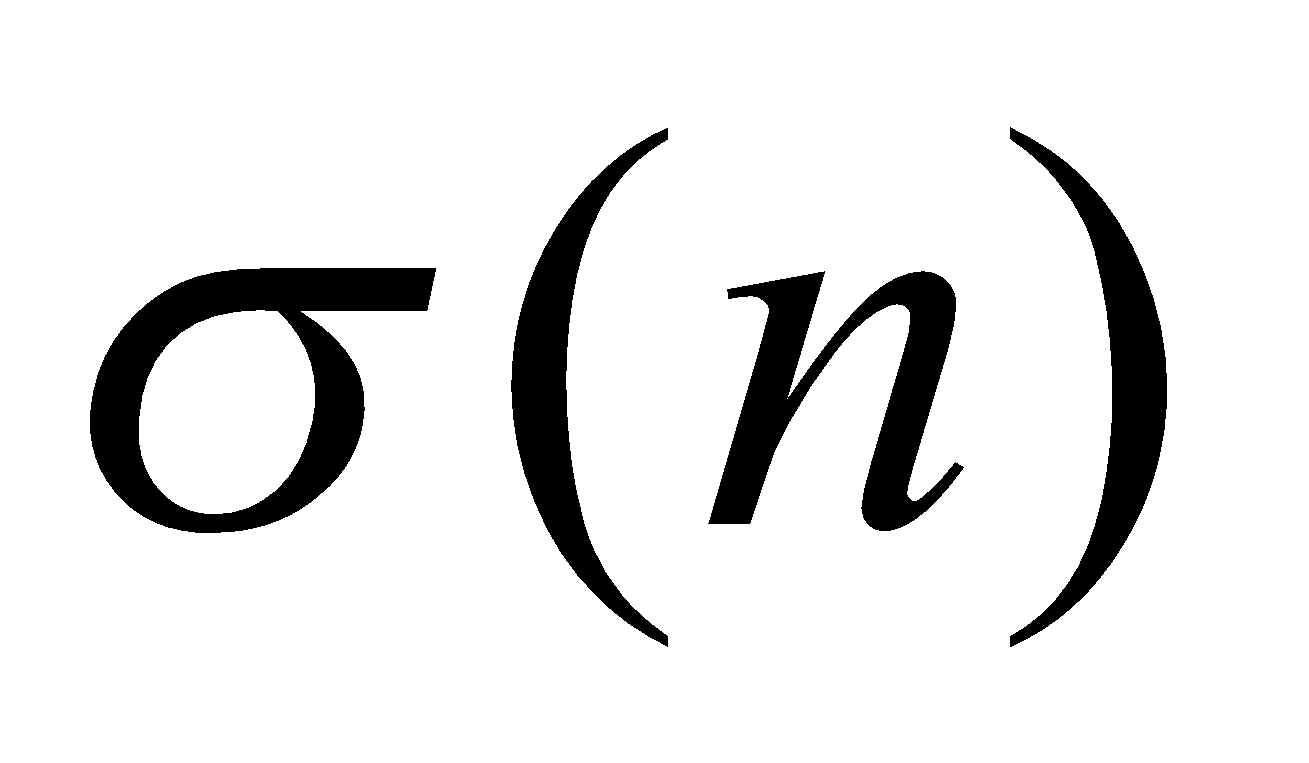defined as the sum of the divisors of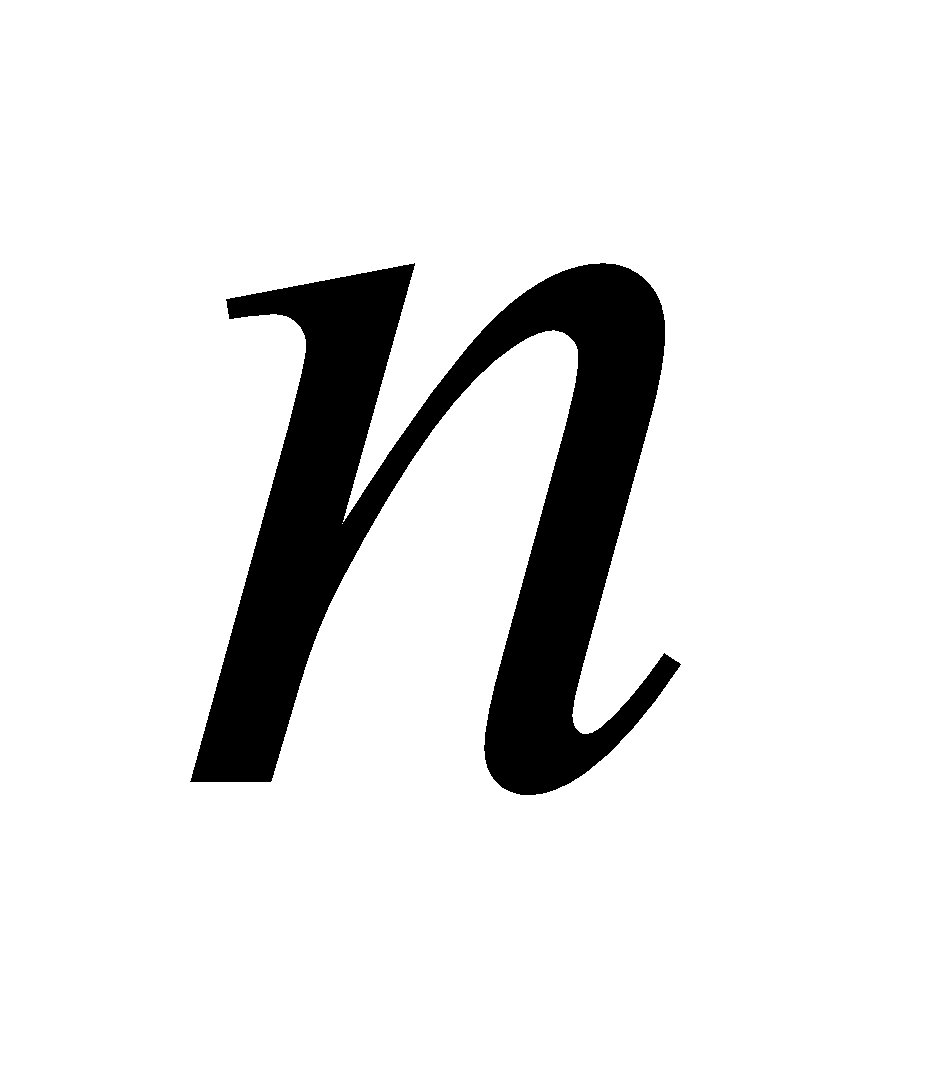, multiplicative?
6. Answer the following questions: 3x6=18
1. Prove that if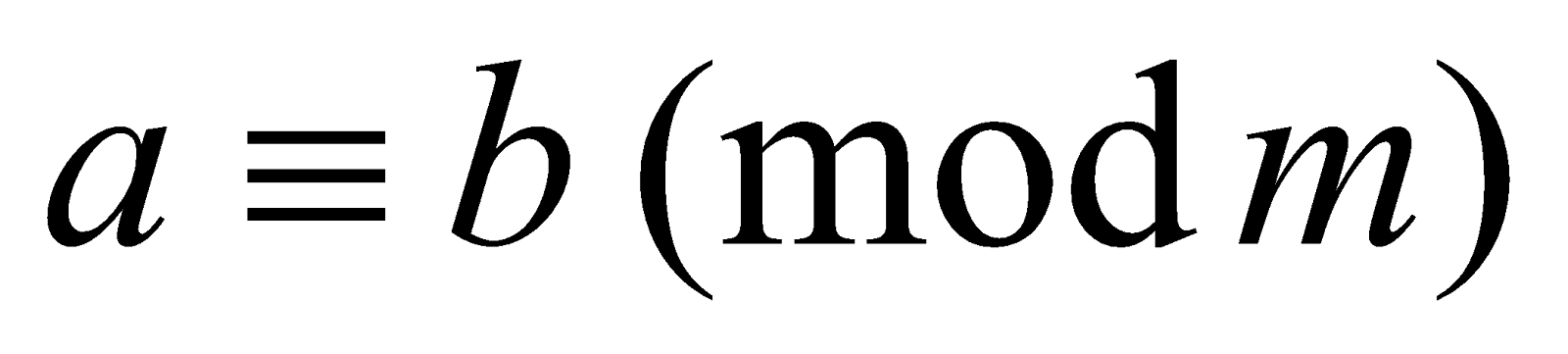and, then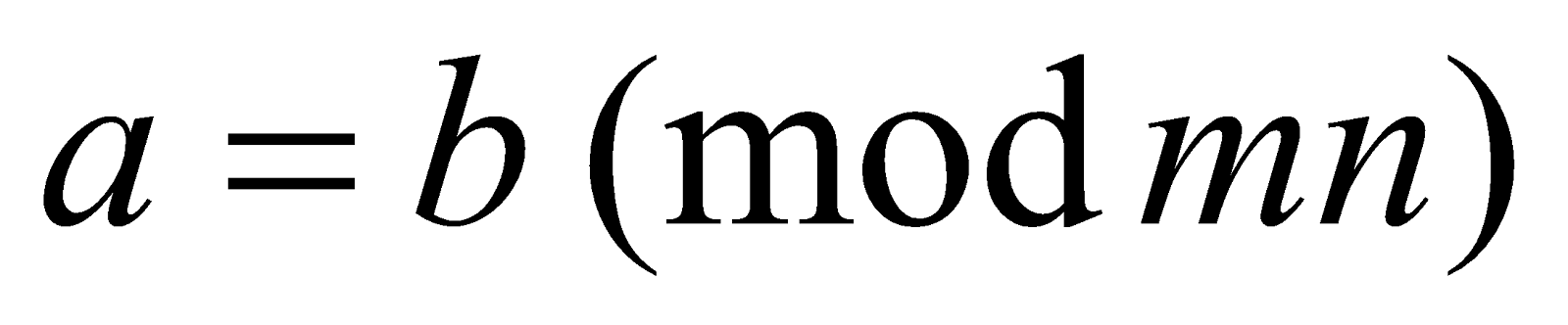1. Solve in integers: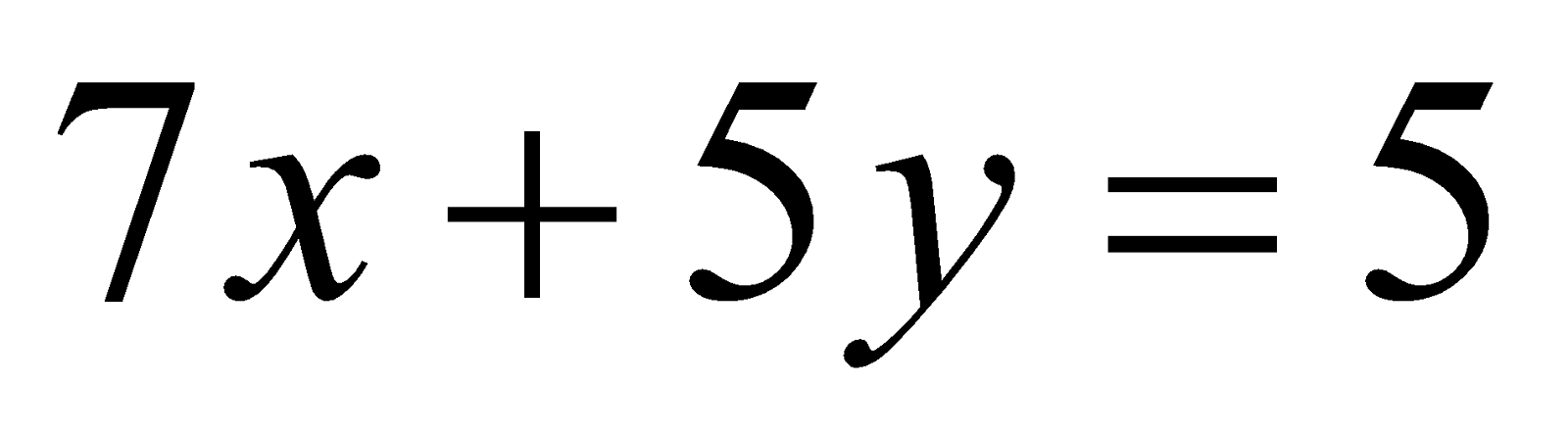1. By the principle of mathematical induction, prove that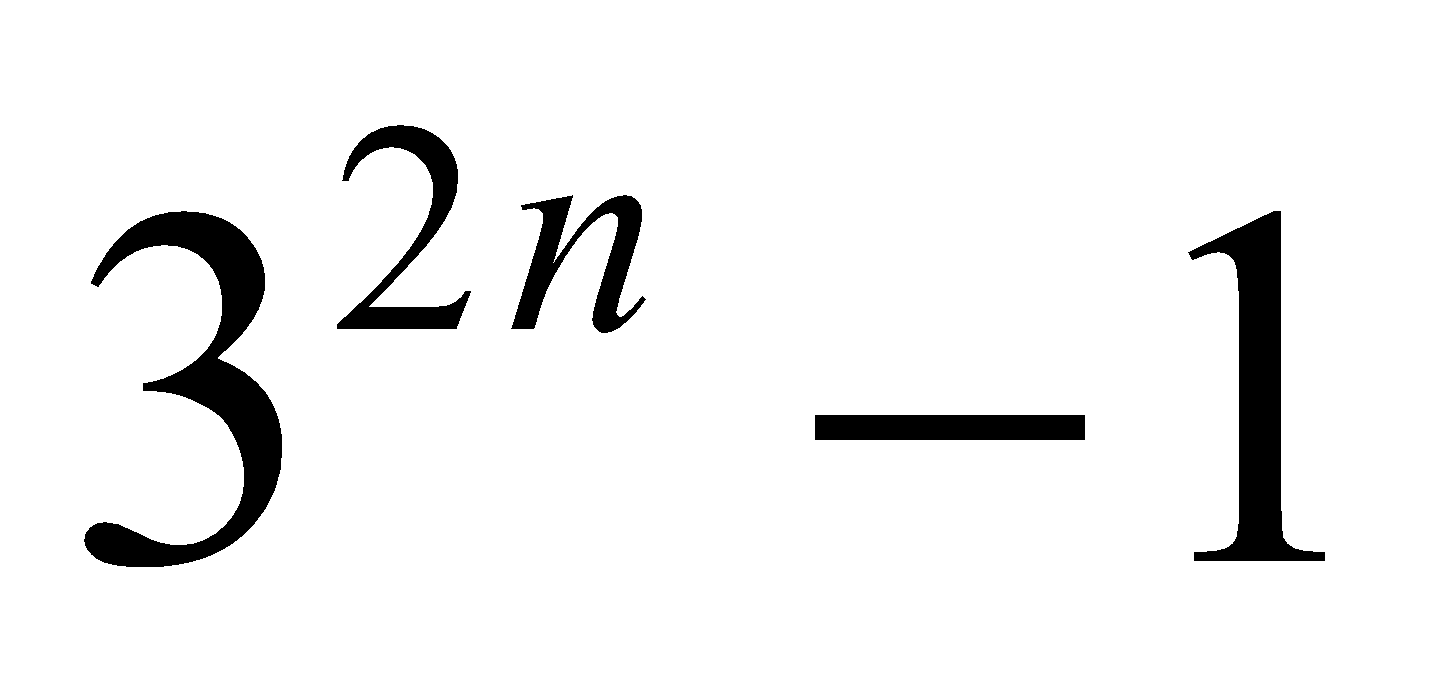is divisible by.
2. Find the highest power of 5 which is contained in 500!.
3. Is the system of linear congruence given below solvable? Give reasons for your answer: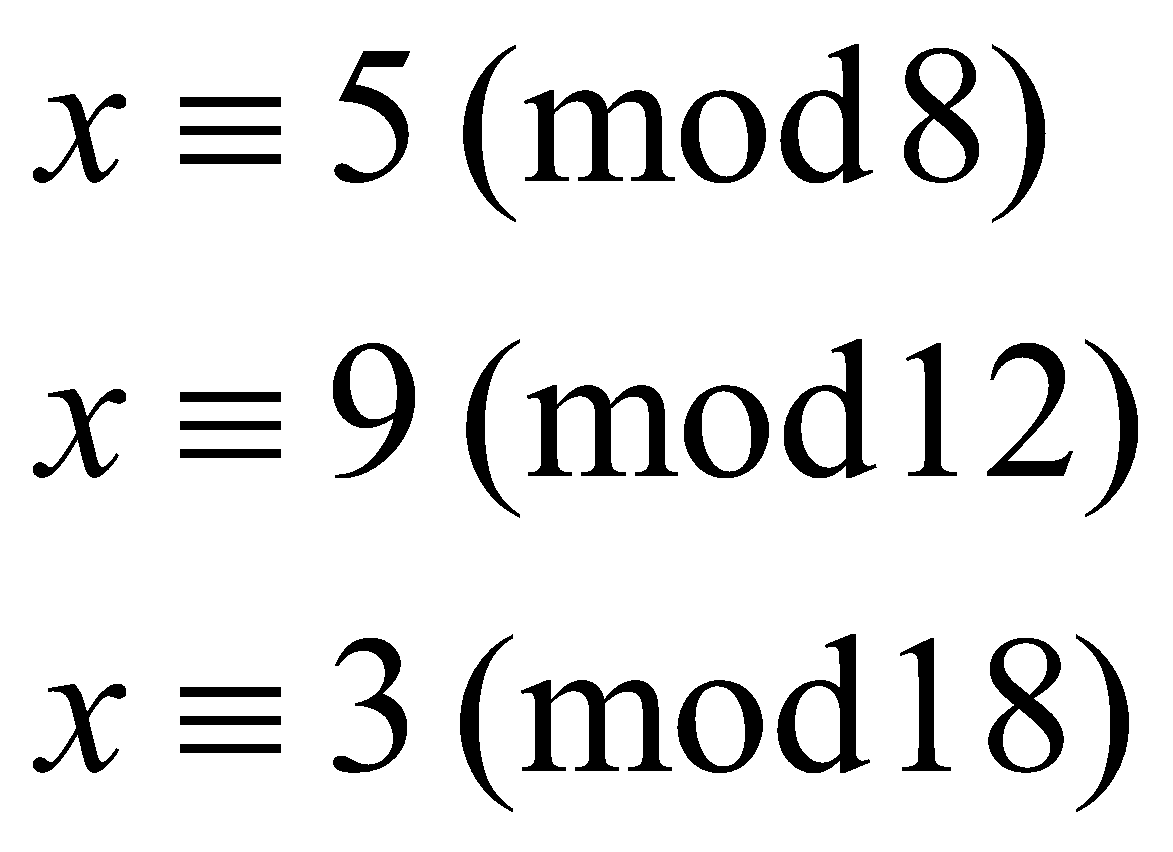1. Find the value of the following:
1.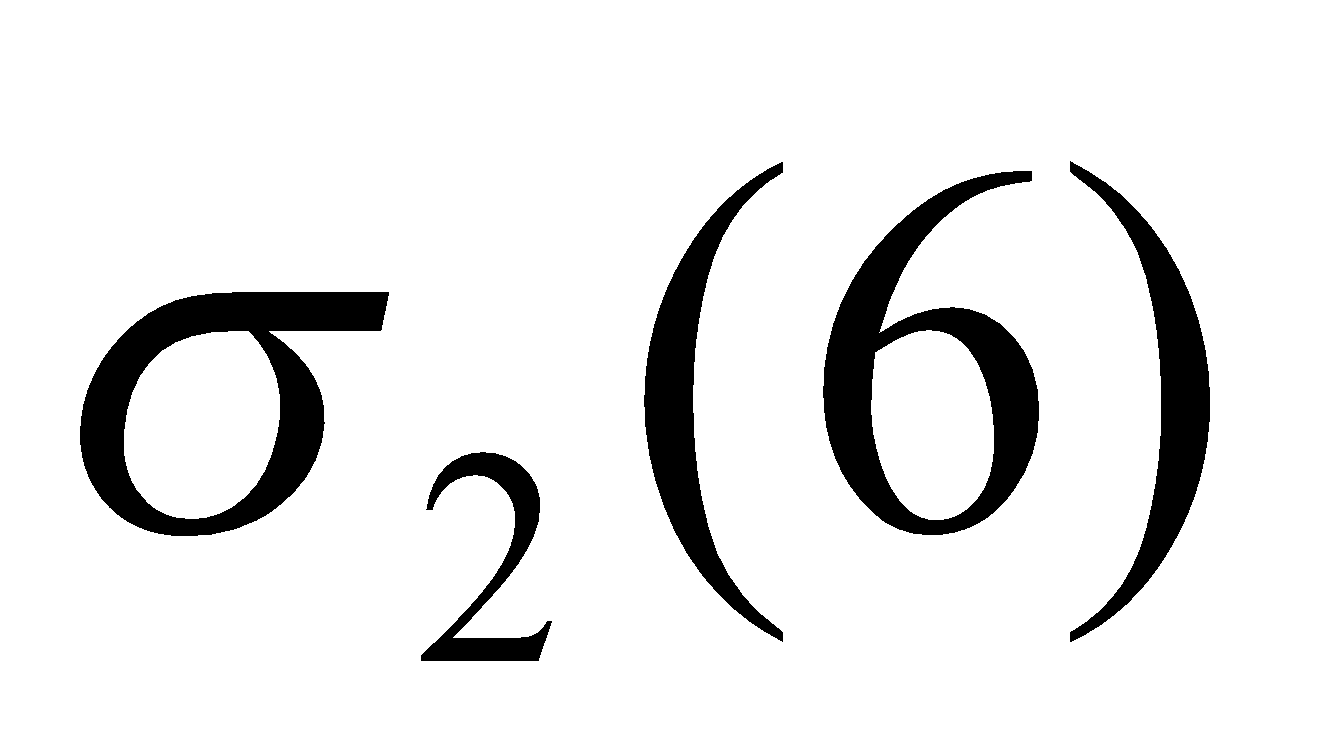2.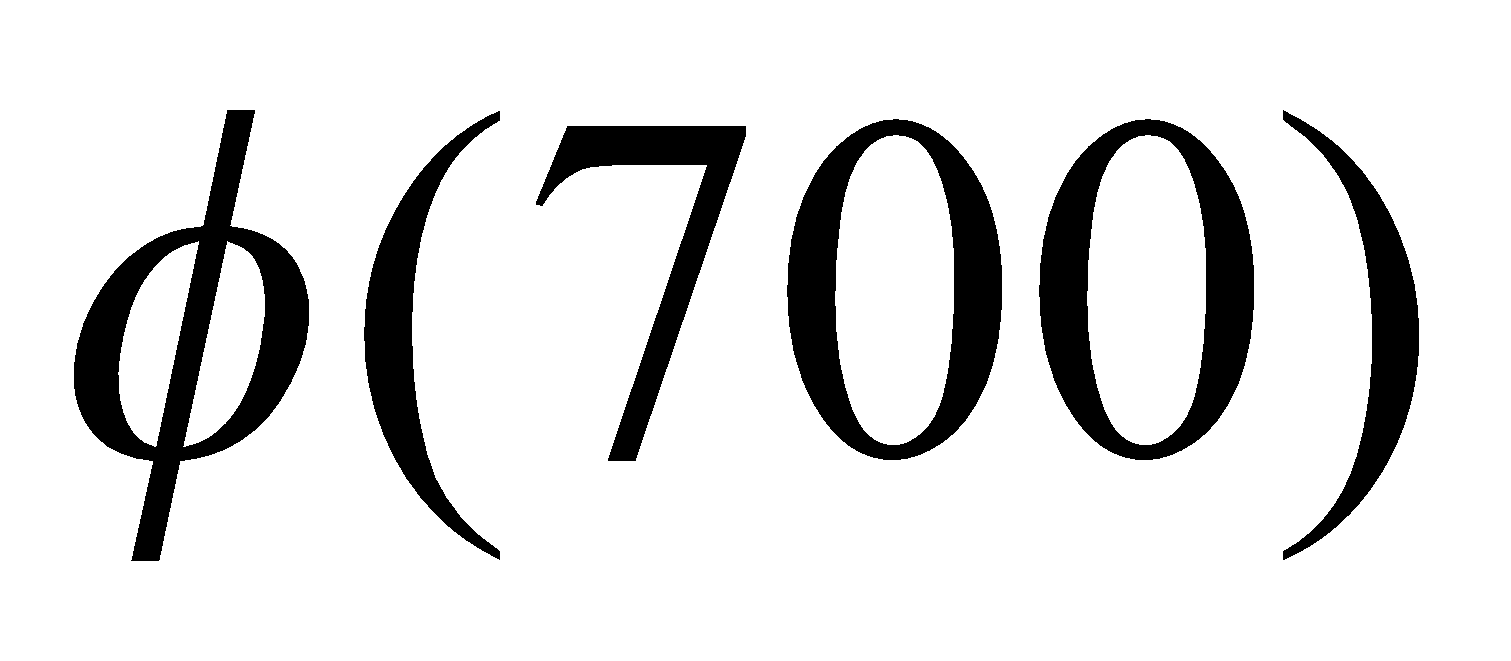7. Answer any three of the following: 4x3=12
1. If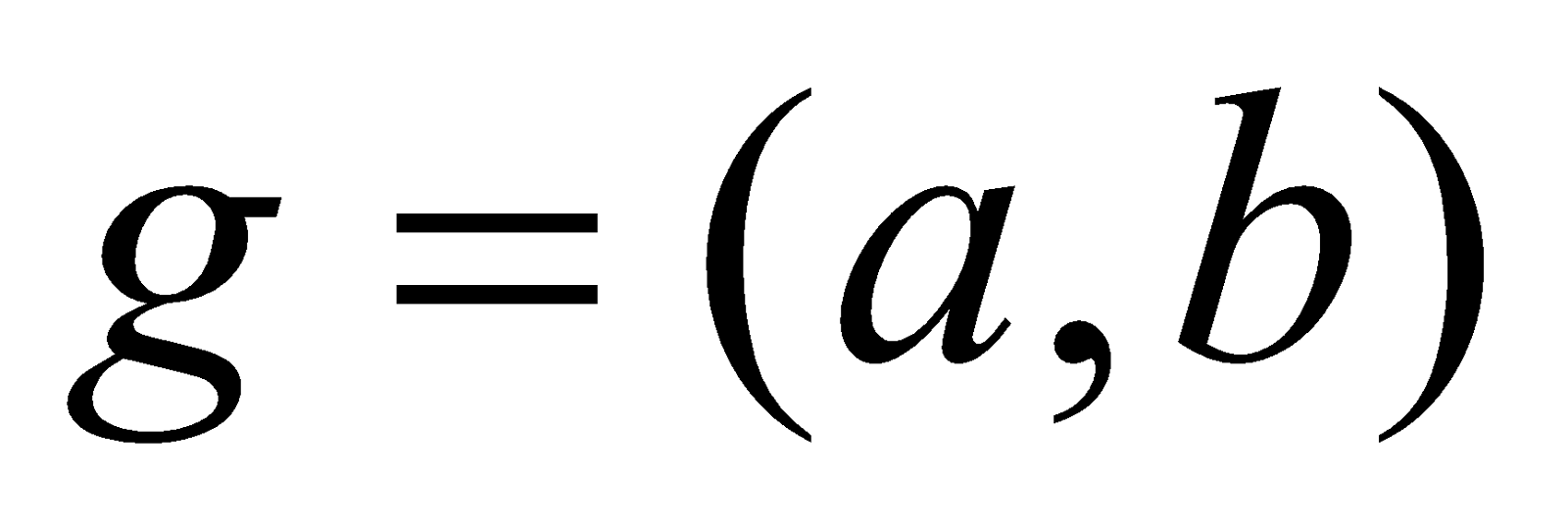, then prove that there exist integers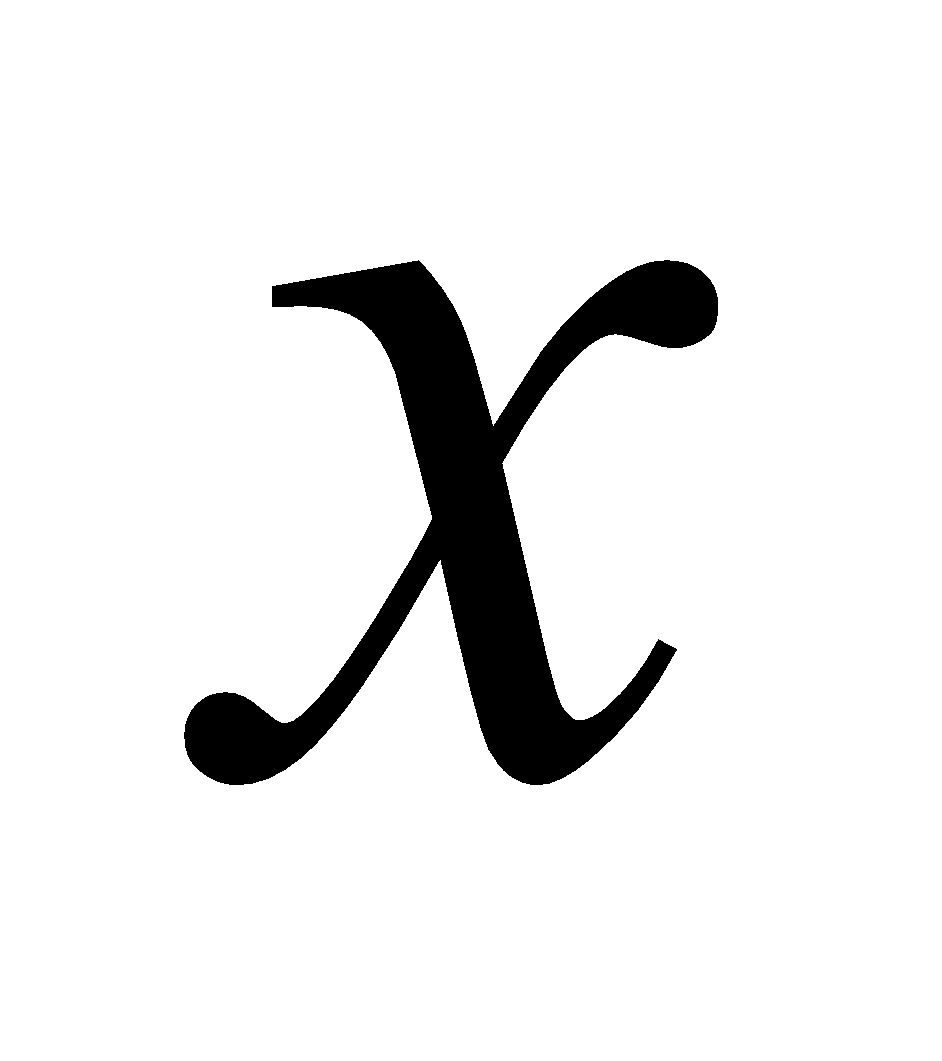and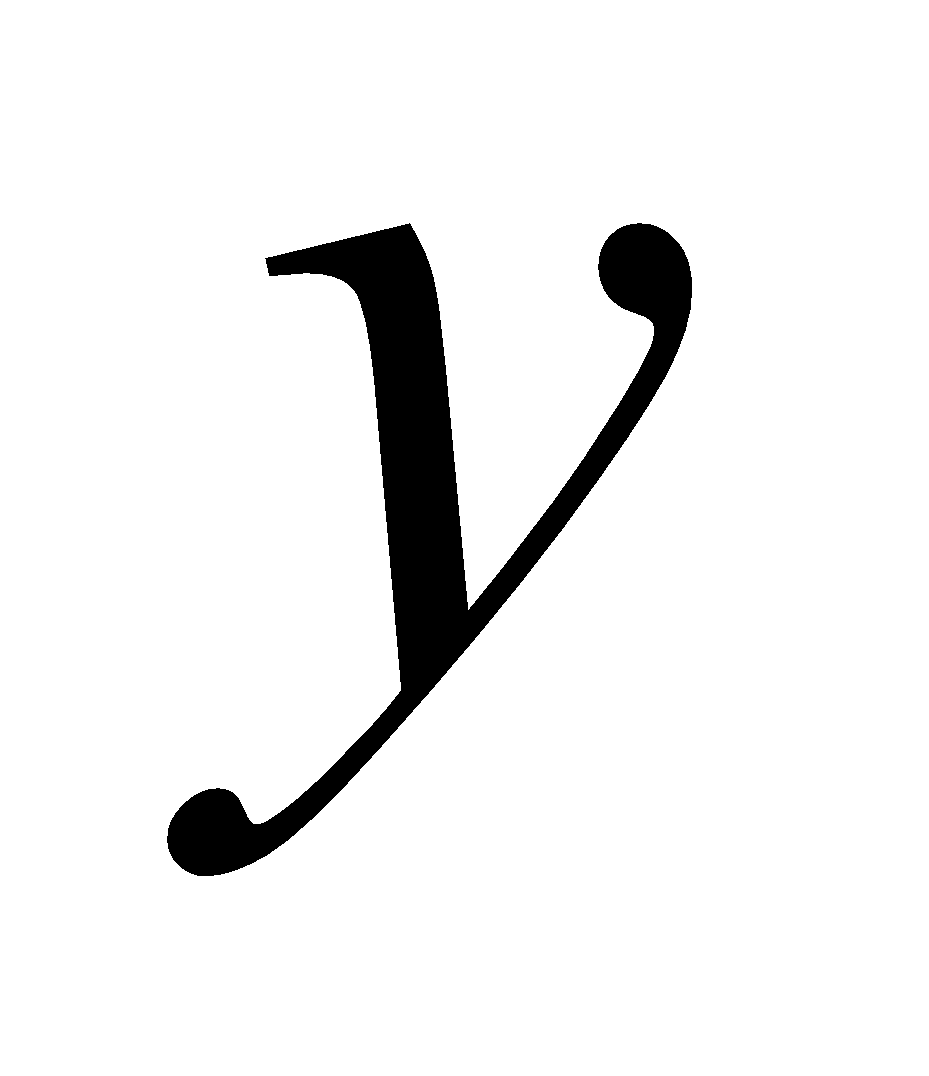such that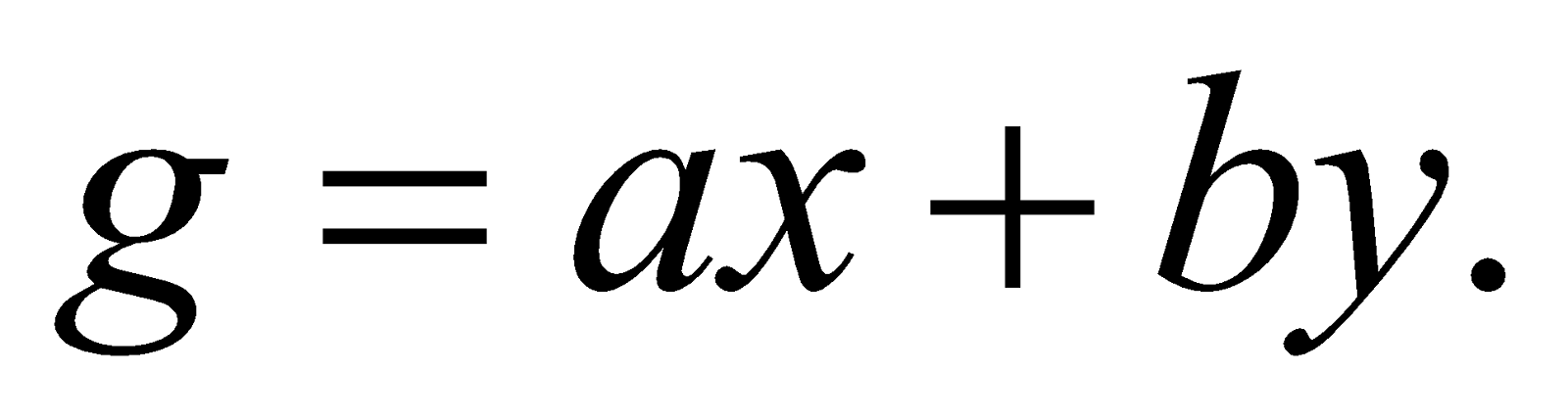2. State Fermat’s little theorem. Using Fermat’s little theorem, find the remainder when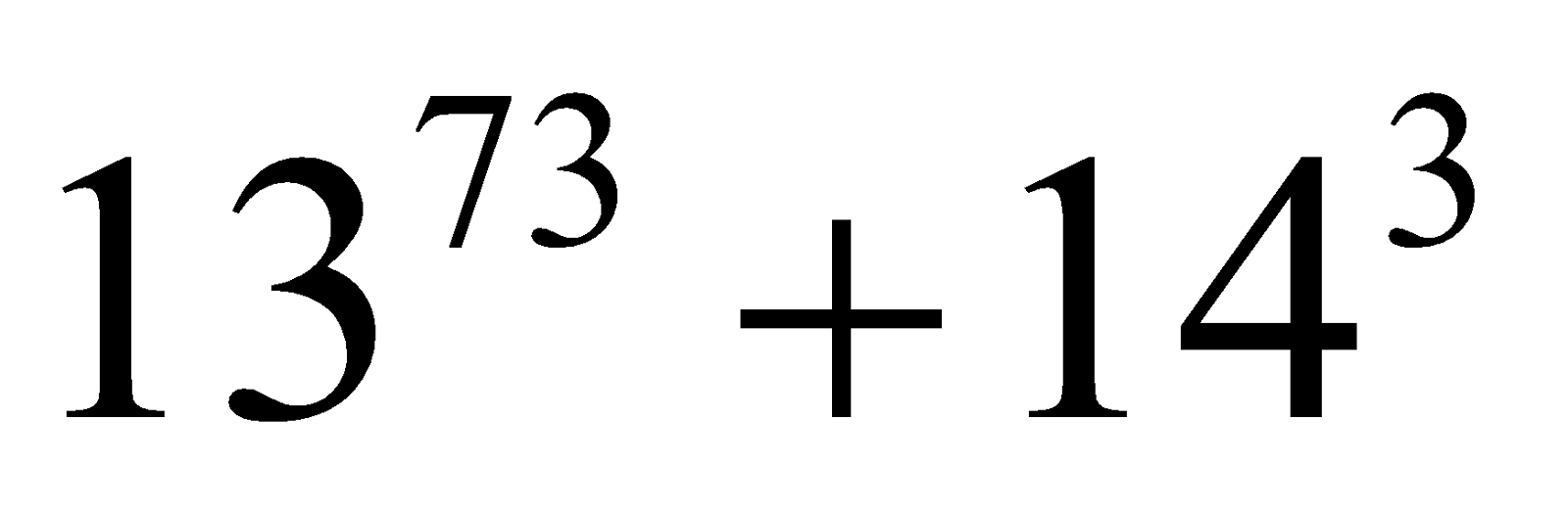is divided by 11.
3. Prove that there are infinitely many primes.
4. Prove that for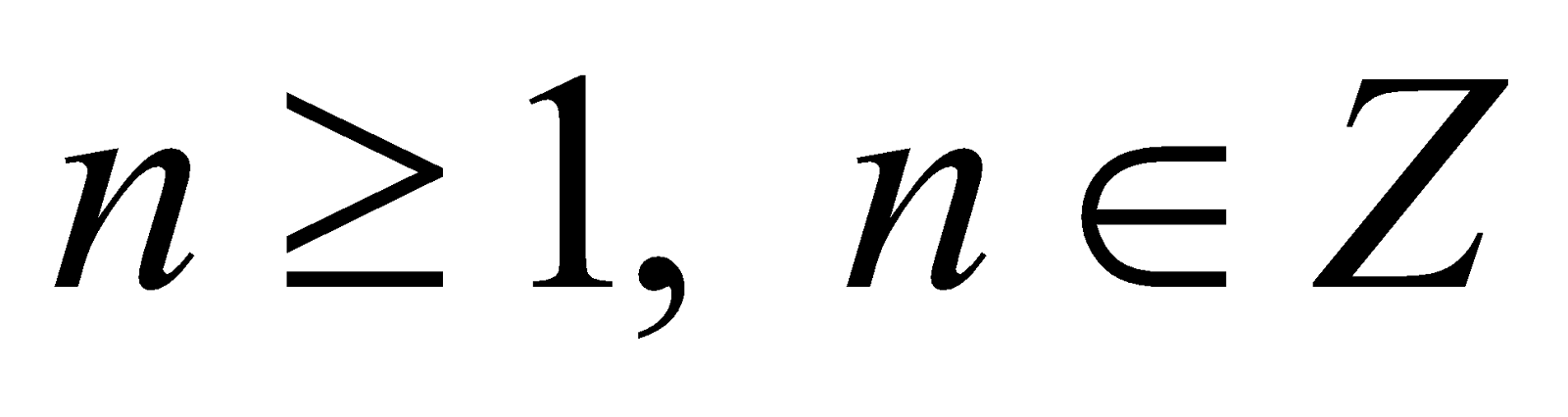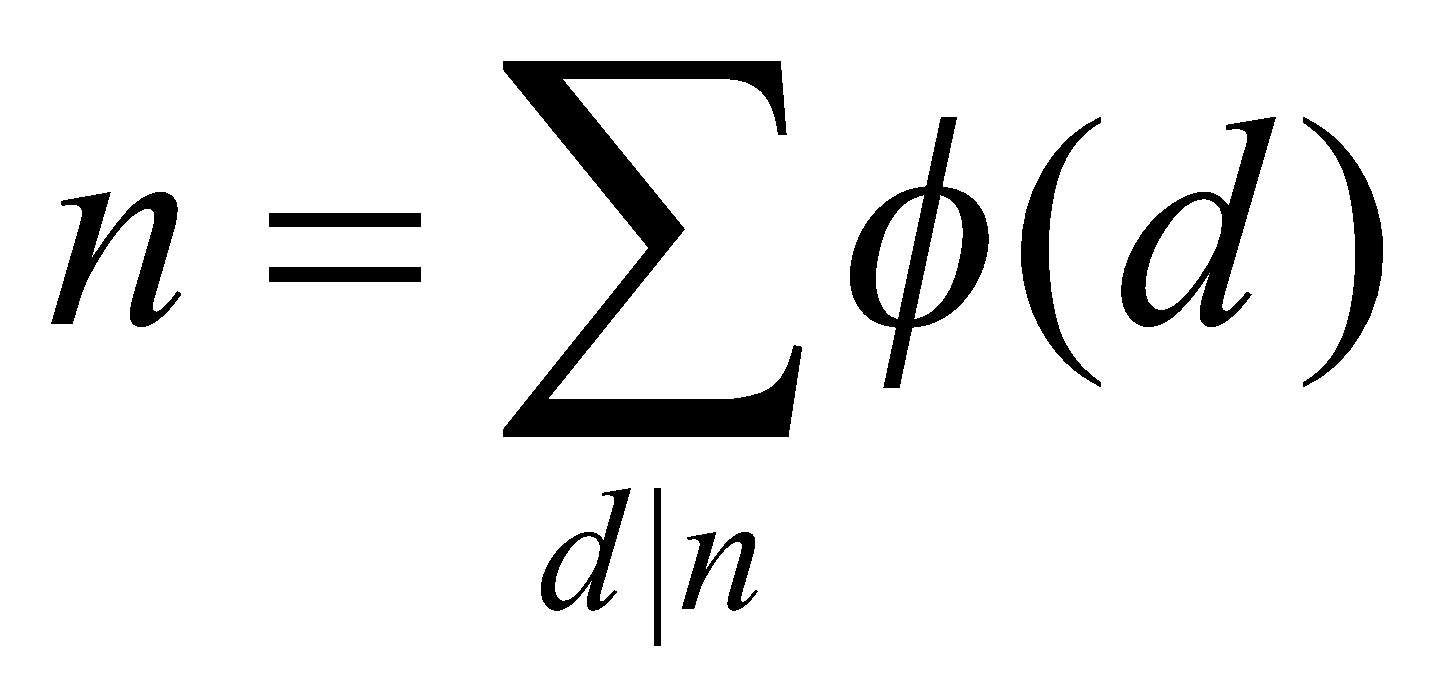***